# US20110059545A1 - Time-temperature indicator based on oligomeric spiroaromatics - Google Patents

## Info

Publication number
US20110059545A1
US20110059545A1 US12/523,110 US52311008A US2011059545A1 US 20110059545 A1 US20110059545 A1 US 20110059545A1 US 52311008 A US52311008 A US 52311008A US 2011059545 A1 US2011059545 A1 US 2011059545A1
Authority
US
United States
Prior art keywords
hydrogen
indicator
alkyl
time
induction
Prior art date
Legal status (The legal status is an assumption and is not a legal conclusion. Google has not performed a legal analysis and makes no representation as to the accuracy of the status listed.)
Abandoned
Application number
US12/523,110
Inventor
Husein Salman
Elena Tenetov
Leonhard Feiler
Thomas Raimann
Current Assignee (The listed assignees may be inaccurate. Google has not performed a legal analysis and makes no representation or warranty as to the accuracy of the list.)
FRESHPOINT HOLDINGS SA
Original Assignee
FRESHPOINT HOLDINGS SA
Priority date (The priority date is an assumption and is not a legal conclusion. Google has not performed a legal analysis and makes no representation as to the accuracy of the date listed.)
Filing date
Publication date
Priority claimed from EP07100873 external-priority
Application filed by FRESHPOINT HOLDINGS SA filed Critical FRESHPOINT HOLDINGS SA
Assigned to FRESHPOINT HOLDINGS S.A. reassignment FRESHPOINT HOLDINGS S.A. ASSIGNMENT OF ASSIGNORS INTEREST (SEE DOCUMENT FOR DETAILS). Assignors: RAIMANN, THOMAS, FEILER, LEONHARD, SALMAN, HUSEIN, TENETOV, ELENA
Publication of US20110059545A1 publication Critical patent/US20110059545A1/en
Abandoned legal-status Critical Current

• 229910052739 hydrogen Inorganic materials 0.000 claims abstract description 56
• 239000001257 hydrogen Substances 0.000 claims abstract description 56
• 125000004435 hydrogen atoms Chemical group [H]* 0.000 claims abstract description 44
• 229910052736 halogen Inorganic materials 0.000 claims abstract description 38
• 150000002367 halogens Chemical class 0.000 claims abstract description 38
• -1 -C1-C6 alkoxy Chemical group 0.000 claims abstract description 30
• 125000004169 (C1-C6) alkyl group Chemical group 0.000 claims abstract description 28
• 125000000449 nitro group Chemical group [O-][N+](*)=O 0.000 claims abstract description 20
• 125000001997 phenyl group Chemical group [H]C1=C([H])C([H])=C(*)C([H])=C1[H] 0.000 claims abstract description 18
• 125000002023 trifluoromethyl group Chemical group FC(F)(F)* 0.000 claims abstract description 18
• 125000003178 carboxy group Chemical group [H]OC(*)=O 0.000 claims abstract description 14
• 150000002431 hydrogen Chemical group 0.000 claims abstract 10
• 150000001875 compounds Chemical class 0.000 claims description 90
• 238000000034 method Methods 0.000 claims description 72
• 230000001939 inductive effect Effects 0.000 claims description 70
• 238000006243 chemical reaction Methods 0.000 claims description 60
• 239000011159 matrix material Substances 0.000 claims description 44
• 239000000126 substance Substances 0.000 claims description 26
• 238000007639 printing Methods 0.000 claims description 24
• 125000000956 methoxy group Chemical group [H]C([H])([H])O* 0.000 claims description 16
• 230000001419 dependent Effects 0.000 claims description 12
• 125000002496 methyl group Chemical group [H]C([H])([H])* 0.000 claims description 12
• 239000012141 concentrate Substances 0.000 claims description 10
• 125000001495 ethyl group Chemical group [H]C([H])([H])C([H])([H])* 0.000 claims description 8
• 238000004519 manufacturing process Methods 0.000 claims description 8
• 239000000049 pigment Substances 0.000 claims description 8
• 230000001012 protector Effects 0.000 claims description 4
• 125000003545 alkoxy group Chemical group 0.000 abstract 2
• 125000000217 alkyl group Chemical group 0.000 abstract 2
• LFQSCWFLJHTTHZ-UHFFFAOYSA-N ethanol Chemical compound CCO LFQSCWFLJHTTHZ-UHFFFAOYSA-N 0.000 description 120
• 239000000976 ink Substances 0.000 description 42
• 239000000203 mixture Substances 0.000 description 42
• YMWUJEATGCHHMB-UHFFFAOYSA-N methylene dichloride Chemical compound ClCCl YMWUJEATGCHHMB-UHFFFAOYSA-N 0.000 description 40
• QTBSBXVTEAMEQO-UHFFFAOYSA-N acetic acid Chemical compound CC(O)=O QTBSBXVTEAMEQO-UHFFFAOYSA-N 0.000 description 36
• 239000011541 reaction mixture Substances 0.000 description 34
• YXFVVABEGXRONW-UHFFFAOYSA-N toluene Chemical compound CC1=CC=CC=C1 YXFVVABEGXRONW-UHFFFAOYSA-N 0.000 description 32
• 239000000047 product Substances 0.000 description 28
• XLYOFNOQVPJJNP-UHFFFAOYSA-N water Substances O XLYOFNOQVPJJNP-UHFFFAOYSA-N 0.000 description 24
• 238000002360 preparation method Methods 0.000 description 22
• UHOVQNZJYSORNB-UHFFFAOYSA-N benzene Chemical compound C1=CC=CC=C1 UHOVQNZJYSORNB-UHFFFAOYSA-N 0.000 description 20
• 239000000243 solution Substances 0.000 description 20
• XEKOWRVHYACXOJ-UHFFFAOYSA-N acetic acid ethyl ester Chemical compound CCOC(C)=O XEKOWRVHYACXOJ-UHFFFAOYSA-N 0.000 description 18
• HEMHJVSKTPXQMS-UHFFFAOYSA-M sodium hydroxide Chemical compound [OH-].[Na+] HEMHJVSKTPXQMS-UHFFFAOYSA-M 0.000 description 18
• 238000005562 fading Methods 0.000 description 16
• WSFSSNUMVMOOMR-UHFFFAOYSA-N formaldehyde Chemical compound O=C WSFSSNUMVMOOMR-UHFFFAOYSA-N 0.000 description 16
• VLKZOEOYAKHREP-UHFFFAOYSA-N hexane Chemical compound CCCCCC VLKZOEOYAKHREP-UHFFFAOYSA-N 0.000 description 16
• 239000010410 layer Substances 0.000 description 16
• IJGRMHOSHXDMSA-UHFFFAOYSA-N nitrogen Chemical compound N#N IJGRMHOSHXDMSA-UHFFFAOYSA-N 0.000 description 16
• 239000002904 solvent Substances 0.000 description 16
• 125000005647 linker group Chemical group 0.000 description 14
• 229920000642 polymer Polymers 0.000 description 14
• BWHMMNNQKKPAPP-UHFFFAOYSA-L potassium carbonate Chemical compound [K+].[K+].[O-]C([O-])=O BWHMMNNQKKPAPP-UHFFFAOYSA-L 0.000 description 14
• PNEYBMLMFCGWSK-UHFFFAOYSA-N AI2O3 Inorganic materials [O-2].[O-2].[O-2].[Al+3].[Al+3] PNEYBMLMFCGWSK-UHFFFAOYSA-N 0.000 description 12
• WEVYAHXRMPXWCK-UHFFFAOYSA-N acetonitrile Chemical compound CC#N WEVYAHXRMPXWCK-UHFFFAOYSA-N 0.000 description 12
• HEDRZPFGACZZDS-UHFFFAOYSA-N chloroform Chemical compound ClC(Cl)Cl HEDRZPFGACZZDS-UHFFFAOYSA-N 0.000 description 12
• 239000012458 free base Substances 0.000 description 12
• 239000000463 material Substances 0.000 description 12
• 238000000655 nuclear magnetic resonance spectrum Methods 0.000 description 12
• CDBYLPFSWZWCQE-UHFFFAOYSA-L sodium carbonate Substances [Na+].[Na+].[O-]C([O-])=O CDBYLPFSWZWCQE-UHFFFAOYSA-L 0.000 description 12
• 239000007787 solid Substances 0.000 description 12
• 238000003756 stirring Methods 0.000 description 12
• DFMPMYDHPGBZCX-UHFFFAOYSA-N 1-[[4-[4-[(3,3-dimethyl-2-methylideneindol-1-yl)methyl]phenyl]phenyl]methyl]-3,3-dimethyl-2-methylideneindole Chemical group C12=CC=CC=C2C(C)(C)C(=C)N1CC1=CC=C(C=2C=CC(CN3C4=CC=CC=C4C(C)(C)C3=C)=CC=2)C=C1 DFMPMYDHPGBZCX-UHFFFAOYSA-N 0.000 description 10
• HIXDQWDOVZUNNA-UHFFFAOYSA-N 2-(3,4-dimethoxyphenyl)-5-hydroxy-7-methoxychromen-4-one Chemical compound C=1C(OC)=CC(O)=C(C(C=2)=O)C=1OC=2C1=CC=C(OC)C(OC)=C1 HIXDQWDOVZUNNA-UHFFFAOYSA-N 0.000 description 10
• URLKBWYHVLBVBO-UHFFFAOYSA-N CC1=CC=C(C)C=C1 Chemical compound CC1=CC=C(C)C=C1 URLKBWYHVLBVBO-UHFFFAOYSA-N 0.000 description 10
• 239000007832 Na2SO4 Substances 0.000 description 10
• 238000001816 cooling Methods 0.000 description 10
• 239000012043 crude product Substances 0.000 description 10
• RTZKZFJDLAIYFH-UHFFFAOYSA-N ether Substances CCOCC RTZKZFJDLAIYFH-UHFFFAOYSA-N 0.000 description 10
• 239000011521 glass Substances 0.000 description 10
• 239000008079 hexane Substances 0.000 description 10
• 239000012074 organic phase Substances 0.000 description 10
• VYPSYNLAJGMNEJ-UHFFFAOYSA-N silicium dioxide Chemical compound O=[Si]=O VYPSYNLAJGMNEJ-UHFFFAOYSA-N 0.000 description 10
• 229910052938 sodium sulfate Inorganic materials 0.000 description 10
• 239000000758 substrate Substances 0.000 description 10
• 0 [1*]C1=C([2*])C([3*])=C([4*])C2=C1OC1(C=C2)N(C*CN2C3=C(C=CC=C3)C(C)(C)C23C=CC2=C(O3)C([1*])=C([2*])C([3*])=C2[4*])C2=CC=CC=C2C1(C)C.[1*]C1=C([2*])C([3*])=C([4*])C2=C1OC1(C=C2)N(CC)C2=C(C=CC=C2)C1(C)C.[1*]C1=C([2*])C([3*])=C([4*])C2=C1OC1(C=C2)N(CC)C2=C(C=CC=C2)C1(C)C.[1*]C1=C([2*])C([3*])=C([4*])C2=C1OC1(C=C2)N(CC)C2=CC=CC=C2C1(C)C.[11*]C.[11*]C.[11*]C.[11*]C.[11*]C.[5*]C.[5*]C.[5*]C.[5*]C.[5*]C Chemical compound [1*]C1=C([2*])C([3*])=C([4*])C2=C1OC1(C=C2)N(C*CN2C3=C(C=CC=C3)C(C)(C)C23C=CC2=C(O3)C([1*])=C([2*])C([3*])=C2[4*])C2=CC=CC=C2C1(C)C.[1*]C1=C([2*])C([3*])=C([4*])C2=C1OC1(C=C2)N(CC)C2=C(C=CC=C2)C1(C)C.[1*]C1=C([2*])C([3*])=C([4*])C2=C1OC1(C=C2)N(CC)C2=C(C=CC=C2)C1(C)C.[1*]C1=C([2*])C([3*])=C([4*])C2=C1OC1(C=C2)N(CC)C2=CC=CC=C2C1(C)C.[11*]C.[11*]C.[11*]C.[11*]C.[11*]C.[5*]C.[5*]C.[5*]C.[5*]C.[5*]C 0.000 description 9
• YJTKZCDBKVTVBY-UHFFFAOYSA-N 1,3-Diphenylbenzene Chemical group C1=CC=CC=C1C1=CC=CC(C=2C=CC=CC=2)=C1 YJTKZCDBKVTVBY-UHFFFAOYSA-N 0.000 description 8
• HMUGRILXVBKBID-UHFFFAOYSA-N 1-(bromomethyl)-4-[4-(bromomethyl)phenyl]benzene Chemical group C1=CC(CBr)=CC=C1C1=CC=C(CBr)C=C1 HMUGRILXVBKBID-UHFFFAOYSA-N 0.000 description 8
• ZWEHNKRNPOVVGH-UHFFFAOYSA-N 2-butanone Chemical compound CCC(C)=O ZWEHNKRNPOVVGH-UHFFFAOYSA-N 0.000 description 8
• 239000011149 active material Substances 0.000 description 8
• 238000009835 boiling Methods 0.000 description 8
• 238000010438 heat treatment Methods 0.000 description 8
• 239000005457 ice water Substances 0.000 description 8
• 238000005259 measurement Methods 0.000 description 8
• 229910052751 metal Inorganic materials 0.000 description 8
• 239000002184 metal Substances 0.000 description 8
• 229910052757 nitrogen Inorganic materials 0.000 description 8
• 229920002866 paraformaldehyde Polymers 0.000 description 8
• 239000001184 potassium carbonate Substances 0.000 description 8
• 230000002829 reduced Effects 0.000 description 8
• 229920005989 resin Polymers 0.000 description 8
• 239000011347 resin Substances 0.000 description 8
• RYHBNJHYFVUHQT-UHFFFAOYSA-N 1,4-dioxane Chemical compound C1COCCO1 RYHBNJHYFVUHQT-UHFFFAOYSA-N 0.000 description 6
• FLHJIAFUWHPJRT-UHFFFAOYSA-N 2,3,3-trimethylindole Chemical compound C1=CC=C2C(C)(C)C(C)=NC2=C1 FLHJIAFUWHPJRT-UHFFFAOYSA-N 0.000 description 6
• 229920000180 Alkyd Polymers 0.000 description 6
• PCLIMKBDDGJMGD-UHFFFAOYSA-N N-Bromosuccinimide Chemical compound BrN1C(=O)CCC1=O PCLIMKBDDGJMGD-UHFFFAOYSA-N 0.000 description 6
• SMQUZDBALVYZAC-UHFFFAOYSA-N Salicylaldehyde Chemical class OC1=CC=CC=C1C=O SMQUZDBALVYZAC-UHFFFAOYSA-N 0.000 description 6
• CSCPPACGZOOCGX-UHFFFAOYSA-N acetone Chemical compound CC(C)=O CSCPPACGZOOCGX-UHFFFAOYSA-N 0.000 description 6
• 238000004458 analytical method Methods 0.000 description 6
• 150000001491 aromatic compounds Chemical class 0.000 description 6
• 239000002585 base Substances 0.000 description 6
• 230000015572 biosynthetic process Effects 0.000 description 6
• SPWVRYZQLGQKGK-UHFFFAOYSA-N dichloromethane;hexane Chemical compound ClCCl.CCCCCC SPWVRYZQLGQKGK-UHFFFAOYSA-N 0.000 description 6
• 239000000975 dye Substances 0.000 description 6
• 238000003475 lamination Methods 0.000 description 6
• GRYLNZFGIOXLOG-UHFFFAOYSA-N nitric acid Chemical class O[N+]([O-])=O GRYLNZFGIOXLOG-UHFFFAOYSA-N 0.000 description 6
• 239000003960 organic solvent Substances 0.000 description 6
• 239000000123 paper Substances 0.000 description 6
• NBIIXXVUZAFLBC-UHFFFAOYSA-N phosphoric acid Chemical compound OP(O)(O)=O NBIIXXVUZAFLBC-UHFFFAOYSA-N 0.000 description 6
• 229910000027 potassium carbonate Inorganic materials 0.000 description 6
• 239000000843 powder Substances 0.000 description 6
• 239000001187 sodium carbonate Substances 0.000 description 6
• 229910000029 sodium carbonate Inorganic materials 0.000 description 6
• 239000002966 varnish Substances 0.000 description 6
• QENIALCDPFDFHX-UHFFFAOYSA-N 1,4-dibromo-2,5-dimethylbenzene Chemical group CC1=CC(Br)=C(C)C=C1Br QENIALCDPFDFHX-UHFFFAOYSA-N 0.000 description 5
• KGKAYWMGPDWLQZ-UHFFFAOYSA-N 1,2-bis(bromomethyl)benzene Chemical group BrCC1=CC=CC=C1CBr KGKAYWMGPDWLQZ-UHFFFAOYSA-N 0.000 description 4
• KLEOTAJNEAAHOP-UHFFFAOYSA-N 1,4-dibromo-2,5-bis(dibromomethyl)benzene Chemical compound BrC(Br)C1=CC(Br)=C(C(Br)Br)C=C1Br KLEOTAJNEAAHOP-UHFFFAOYSA-N 0.000 description 4
• FXMONWISTWQYFY-UHFFFAOYSA-N 1-[[2,5-dibromo-4-[(3,3-dimethyl-2-methylideneindol-1-yl)methyl]phenyl]methyl]-3,3-dimethyl-2-methylideneindole Chemical compound C12=CC=CC=C2C(C)(C)C(=C)N1CC(C(=C1)Br)=CC(Br)=C1CN1C2=CC=CC=C2C(C)(C)C1=C FXMONWISTWQYFY-UHFFFAOYSA-N 0.000 description 4
• XYBLTSMTRKRRPW-UHFFFAOYSA-N 1-[[4-[(3,3-dimethyl-2-methylideneindol-1-yl)methyl]phenyl]methyl]-3,3-dimethyl-2-methylideneindole Chemical compound C12=CC=CC=C2C(C)(C)C(=C)N1CC(C=C1)=CC=C1CN1C2=CC=CC=C2C(C)(C)C1=C XYBLTSMTRKRRPW-UHFFFAOYSA-N 0.000 description 4
• OWAVOXXCWOKMMB-UHFFFAOYSA-N 1-[[4-[(5-carboxy-3,3-dimethyl-2-methylideneindol-1-yl)methyl]phenyl]methyl]-3,3-dimethyl-2-methylideneindole-5-carboxylic acid Chemical compound C12=CC=C(C(O)=O)C=C2C(C)(C)C(=C)N1CC(C=C1)=CC=C1CN1C2=CC=C(C(O)=O)C=C2C(C)(C)C1=C OWAVOXXCWOKMMB-UHFFFAOYSA-N 0.000 description 4
• MMIOOQCWNGKTIW-UHFFFAOYSA-N 1-[[4-[4-[(3,3-dimethyl-2-methylideneindol-1-yl)methyl]phenyl]phenyl]methyl]-3,3-dimethyl-2-methylideneindole;dihydrobromide Chemical compound Br.Br.C12=CC=CC=C2C(C)(C)C(=C)N1CC1=CC=C(C=2C=CC(CN3C4=CC=CC=C4C(C)(C)C3=C)=CC=2)C=C1 MMIOOQCWNGKTIW-UHFFFAOYSA-N 0.000 description 4
• QOAOUAVSJYANAZ-UHFFFAOYSA-N 1-[[5-[(3,3-dimethyl-2-methylideneindol-1-yl)methyl]naphthalen-1-yl]methyl]-3,3-dimethyl-2-methylideneindole Chemical compound C12=CC=CC=C2C(C)(C)C(=C)N1CC(C1=CC=C2)=CC=CC1=C2CN1C2=CC=CC=C2C(C)(C)C1=C QOAOUAVSJYANAZ-UHFFFAOYSA-N 0.000 description 4
• ZZTFJNNLOBLOPU-UHFFFAOYSA-N 2-hydroxy-3,4-dimethoxy-5-nitrobenzaldehyde Chemical compound COC1=C(O)C(C=O)=CC([N+]([O-])=O)=C1OC ZZTFJNNLOBLOPU-UHFFFAOYSA-N 0.000 description 4
• RZTDESRVPFKCBH-UHFFFAOYSA-N CC1=CC=C(C2=CC=C(C)C=C2)C=C1 Chemical compound CC1=CC=C(C2=CC=C(C)C=C2)C=C1 RZTDESRVPFKCBH-UHFFFAOYSA-N 0.000 description 4
• VZGDMQKNWNREIO-UHFFFAOYSA-N Carbon tetrachloride Chemical compound ClC(Cl)(Cl)Cl VZGDMQKNWNREIO-UHFFFAOYSA-N 0.000 description 4
• 229920000877 Melamine resin Polymers 0.000 description 4
• ZMANZCXQSJIPKH-UHFFFAOYSA-N N,N-Diethylethanamine Substances CCN(CC)CC ZMANZCXQSJIPKH-UHFFFAOYSA-N 0.000 description 4
• JGFZNNIVVJXRND-UHFFFAOYSA-N N,N-Diisopropylethylamine Chemical compound CCN(C(C)C)C(C)C JGFZNNIVVJXRND-UHFFFAOYSA-N 0.000 description 4
• 239000000020 Nitrocellulose Substances 0.000 description 4
• 239000004743 Polypropylene Substances 0.000 description 4
• GSEJCLTVZPLZKY-UHFFFAOYSA-N Tris Chemical compound OCCN(CCO)CCO GSEJCLTVZPLZKY-UHFFFAOYSA-N 0.000 description 4
• 239000000853 adhesive Substances 0.000 description 4
• 239000011230 binding agent Substances 0.000 description 4
• 235000010290 biphenyl Nutrition 0.000 description 4
• 229920002678 cellulose Polymers 0.000 description 4
• 239000003086 colorant Substances 0.000 description 4
• 239000007859 condensation product Substances 0.000 description 4
• 229920001577 copolymer Polymers 0.000 description 4
• 230000000875 corresponding Effects 0.000 description 4
• ZUOUZKKEUPVFJK-UHFFFAOYSA-N diphenyl Chemical compound C1=CC=CC=C1C1=CC=CC=C1 ZUOUZKKEUPVFJK-UHFFFAOYSA-N 0.000 description 4
• 230000000694 effects Effects 0.000 description 4
• 229920001971 elastomer Polymers 0.000 description 4
• 238000001914 filtration Methods 0.000 description 4
• 238000005755 formation reaction Methods 0.000 description 4
• CPELXLSAUQHCOX-UHFFFAOYSA-N hydrogen bromide Chemical compound Br CPELXLSAUQHCOX-UHFFFAOYSA-N 0.000 description 4
• RKJUIXBNRJVNHR-UHFFFAOYSA-N indolenine group Chemical group N1=CCC2=CC=CC=C12 RKJUIXBNRJVNHR-UHFFFAOYSA-N 0.000 description 4
• 150000007529 inorganic bases Chemical class 0.000 description 4
• 230000002427 irreversible Effects 0.000 description 4
• 238000006317 isomerization reaction Methods 0.000 description 4
• 239000007788 liquid Substances 0.000 description 4
• 239000004530 micro-emulsion Substances 0.000 description 4
• 125000000896 monocarboxylic acid group Chemical group 0.000 description 4
• LRHPLDYGYMQRHN-UHFFFAOYSA-N n-butanol Chemical compound CCCCO LRHPLDYGYMQRHN-UHFFFAOYSA-N 0.000 description 4
• 229920001220 nitrocellulos Polymers 0.000 description 4
• 239000005022 packaging material Substances 0.000 description 4
• 238000004806 packaging method and process Methods 0.000 description 4
• 238000007649 pad printing Methods 0.000 description 4
• 238000006068 polycondensation reaction Methods 0.000 description 4
• 229920000728 polyester Polymers 0.000 description 4
• 229920000098 polyolefin Polymers 0.000 description 4
• 229920001296 polysiloxane Polymers 0.000 description 4
• 230000001681 protective Effects 0.000 description 4
• 230000002441 reversible Effects 0.000 description 4
• 239000005060 rubber Substances 0.000 description 4
• 239000000377 silicon dioxide Substances 0.000 description 4
• FAPWRFPIFSIZLT-UHFFFAOYSA-M sodium chloride Chemical compound [Na+].[Cl-] FAPWRFPIFSIZLT-UHFFFAOYSA-M 0.000 description 4
• 239000012265 solid product Substances 0.000 description 4
• 150000003413 spiro compounds Chemical class 0.000 description 4
• PPBRXRYQALVLMV-UHFFFAOYSA-N styrene Chemical compound C=CC1=CC=CC=C1 PPBRXRYQALVLMV-UHFFFAOYSA-N 0.000 description 4
• 125000001424 substituent group Chemical group 0.000 description 4
• 239000000725 suspension Substances 0.000 description 4
• 238000003786 synthesis reaction Methods 0.000 description 4
• WYURNTSHIVDZCO-UHFFFAOYSA-N tetrahydrofuran Chemical compound C1CCOC1 WYURNTSHIVDZCO-UHFFFAOYSA-N 0.000 description 4
• 238000010023 transfer printing Methods 0.000 description 4
• XSQUKJJJFZCRTK-UHFFFAOYSA-N urea Chemical compound NC(N)=O XSQUKJJJFZCRTK-UHFFFAOYSA-N 0.000 description 4
• SDDBCEWUYXVGCQ-UHFFFAOYSA-N 1,5-dimethylnaphthalene Chemical compound C1=CC=C2C(C)=CC=CC2=C1C SDDBCEWUYXVGCQ-UHFFFAOYSA-N 0.000 description 3
• 125000004191 (C1-C6) alkoxy group Chemical group 0.000 description 2
• XJKSTNDFUHDPQJ-UHFFFAOYSA-N 1,4-diphenylbenzene Chemical group C1=CC=CC=C1C1=CC=C(C=2C=CC=CC=2)C=C1 XJKSTNDFUHDPQJ-UHFFFAOYSA-N 0.000 description 2
• ZNHUYTIFYQXOCM-UHFFFAOYSA-N 1,5-bis(bromomethyl)naphthalene Chemical compound C1=CC=C2C(CBr)=CC=CC2=C1CBr ZNHUYTIFYQXOCM-UHFFFAOYSA-N 0.000 description 2
• CZYAFTZIQWCKOI-UHFFFAOYSA-N 1,5-dibromonaphthalene Chemical compound C1=CC=C2C(Br)=CC=CC2=C1Br CZYAFTZIQWCKOI-UHFFFAOYSA-N 0.000 description 2
• RAFPAMKGHLWOKC-UHFFFAOYSA-N 1-[[4-[(3,3-dimethyl-2-methylideneindol-1-yl)methyl]phenyl]methyl]-3,3-dimethyl-2-methylideneindole;dihydrobromide Chemical compound Br.Br.C12=CC=CC=C2C(C)(C)C(=C)N1CC(C=C1)=CC=C1CN1C2=CC=CC=C2C(C)(C)C1=C RAFPAMKGHLWOKC-UHFFFAOYSA-N 0.000 description 2
• HQRKONWTKBYZOA-UHFFFAOYSA-N 2-(4-methylphenyl)-2H-pyran Chemical compound C1=CC(C)=CC=C1C1C=CC=CO1 HQRKONWTKBYZOA-UHFFFAOYSA-N 0.000 description 2
• OXOBKZZXZVFOBB-UHFFFAOYSA-N 2-Diphenylmethylpyrrolidine Chemical compound C1CCNC1C(C=1C=CC=CC=1)C1=CC=CC=C1 OXOBKZZXZVFOBB-UHFFFAOYSA-N 0.000 description 2
• AFABGHUZZDYHJO-UHFFFAOYSA-N 2-Methylpentane Chemical class CCCC(C)C AFABGHUZZDYHJO-UHFFFAOYSA-N 0.000 description 2
• IJVRPNIWWODHHA-UHFFFAOYSA-M 2-cyanoprop-2-enoate Chemical compound [O-]C(=O)C(=C)C#N IJVRPNIWWODHHA-UHFFFAOYSA-M 0.000 description 2
• UIOMNPYQUBMBOJ-UHFFFAOYSA-N 2-hydroxy-3,4-dimethoxybenzaldehyde Chemical compound COC1=CC=C(C=O)C(O)=C1OC UIOMNPYQUBMBOJ-UHFFFAOYSA-N 0.000 description 2
• HGKHVFKBOHFYSS-UHFFFAOYSA-N 2-hydroxy-3-methoxy-5-nitrobenzaldehyde Chemical compound COC1=CC([N+]([O-])=O)=CC(C=O)=C1O HGKHVFKBOHFYSS-UHFFFAOYSA-N 0.000 description 2
• WEYTUUBWDDEAGG-UHFFFAOYSA-N 3,4-dihydro-1H-2,3-benzoxazin-7-amine Chemical compound C1NOCC2=CC(N)=CC=C21 WEYTUUBWDDEAGG-UHFFFAOYSA-N 0.000 description 2
• PCNFLKVWBDNNOW-UHFFFAOYSA-N 4-hydrazinylbenzoic acid Chemical compound NNC1=CC=C(C(O)=O)C=C1 PCNFLKVWBDNNOW-UHFFFAOYSA-N 0.000 description 2
• 229920000178 Acrylic resin Polymers 0.000 description 2
• 239000004925 Acrylic resin Substances 0.000 description 2
• RWCCWEUUXYIKHB-UHFFFAOYSA-N Benzophenone Chemical compound C=1C=CC=CC=1C(=O)C1=CC=CC=C1 RWCCWEUUXYIKHB-UHFFFAOYSA-N 0.000 description 2
• UTGSRNVBAFCOEU-UHFFFAOYSA-N CC1=CC(Cl)=C(C)C=C1Cl Chemical compound CC1=CC(Cl)=C(C)C=C1Cl UTGSRNVBAFCOEU-UHFFFAOYSA-N 0.000 description 2
• GTAGBLIBQSOQHG-UHFFFAOYSA-N COC1=CC(C)=C(OC)C=C1C Chemical compound COC1=CC(C)=C(OC)C=C1C GTAGBLIBQSOQHG-UHFFFAOYSA-N 0.000 description 2
• UTEMSEYMPWBKTR-UHFFFAOYSA-N COC1=CC(CN2C3=CC=CC=C3C(C)(C)C23C=CC2=C(O3)C(C)=CC([N+](=O)[O-])=C2)=C(C)C=C1CN1C2=C(C=CC=C2)C(C)(C)C12C=CC1=C(O2)C(OC)=CC([N+](=O)[O-])=C1 Chemical compound COC1=CC(CN2C3=CC=CC=C3C(C)(C)C23C=CC2=C(O3)C(C)=CC([N+](=O)[O-])=C2)=C(C)C=C1CN1C2=C(C=CC=C2)C(C)(C)C12C=CC1=C(O2)C(OC)=CC([N+](=O)[O-])=C1 UTEMSEYMPWBKTR-UHFFFAOYSA-N 0.000 description 2
• MOGOTJCSXZTBDQ-UHFFFAOYSA-N COC1=CC([N+](=O)[O-])=CC2=C1OC1(/C=C\2)N(CC2=CC=CC3=C(CN4C5=C(C=CC=C5)C(C)(C)C45/C=C\C4=C(O5)C(OC)=CC([N+](=O)[O-])=C4)C=CC=C23)C2=C(C=CC=C2)C1(C)C Chemical compound COC1=CC([N+](=O)[O-])=CC2=C1OC1(/C=C\2)N(CC2=CC=CC3=C(CN4C5=C(C=CC=C5)C(C)(C)C45/C=C\C4=C(O5)C(OC)=CC([N+](=O)[O-])=C4)C=CC=C23)C2=C(C=CC=C2)C1(C)C MOGOTJCSXZTBDQ-UHFFFAOYSA-N 0.000 description 2
• CXNZWXFTNOTPEU-UHFFFAOYSA-N COC1=CC([N+](=O)[O-])=CC2=C1OC1(C=C2)N(CC2=CC=C(C3=CC=C(C4=CC=C(CN5C6=C(C=CC=C6)C(C)(C)C56C=CC5=C(O6)C(OC)=CC([N+](=O)[O-])=C5)C=C4)C=C3)C=C2)C2=CC=CC=C2C1(C)C Chemical compound COC1=CC([N+](=O)[O-])=CC2=C1OC1(C=C2)N(CC2=CC=C(C3=CC=C(C4=CC=C(CN5C6=C(C=CC=C6)C(C)(C)C56C=CC5=C(O6)C(OC)=CC([N+](=O)[O-])=C5)C=C4)C=C3)C=C2)C2=CC=CC=C2C1(C)C CXNZWXFTNOTPEU-UHFFFAOYSA-N 0.000 description 2
• WGCAQULKEDPTGA-UHFFFAOYSA-N COC1=CC([N+](=O)[O-])=CC2=C1OC1(C=C2)N(CC2=CC=C(C3=CC=C(CN4C5=C(C=CC=C5)C(C)(C)C45C=CC4=C(O5)C(OC)=CC([N+](=O)[O-])=C4)C=C3)C=C2)C2=CC=CC=C2C1(C)C Chemical compound COC1=CC([N+](=O)[O-])=CC2=C1OC1(C=C2)N(CC2=CC=C(C3=CC=C(CN4C5=C(C=CC=C5)C(C)(C)C45C=CC4=C(O5)C(OC)=CC([N+](=O)[O-])=C4)C=C3)C=C2)C2=CC=CC=C2C1(C)C WGCAQULKEDPTGA-UHFFFAOYSA-N 0.000 description 2
• 229920002301 Cellulose acetate Polymers 0.000 description 2
• VEXZGXHMUGYJMC-UHFFFAOYSA-N HCl Chemical compound Cl VEXZGXHMUGYJMC-UHFFFAOYSA-N 0.000 description 2
• OMPJBNCRMGITSC-UHFFFAOYSA-N Incidol Chemical compound C=1C=CC=CC=1C(=O)OOC(=O)C1=CC=CC=C1 OMPJBNCRMGITSC-UHFFFAOYSA-N 0.000 description 2
• JDSHMPZPIAZGSV-UHFFFAOYSA-N Melamine Chemical compound NC1=NC(N)=NC(N)=N1 JDSHMPZPIAZGSV-UHFFFAOYSA-N 0.000 description 2
• SYBYTAAJFKOIEJ-UHFFFAOYSA-N Methyl isopropyl ketone Chemical compound CC(C)C(C)=O SYBYTAAJFKOIEJ-UHFFFAOYSA-N 0.000 description 2
• FTWUXYZHDFCGSV-UHFFFAOYSA-N N,N'-diphenyloxamide Chemical compound C=1C=CC=CC=1NC(=O)C(=O)NC1=CC=CC=C1 FTWUXYZHDFCGSV-UHFFFAOYSA-N 0.000 description 2
• ZCILGMFPJBRCNO-UHFFFAOYSA-N OC1=CC=C2NN=NC2=C1C1=CC=CC=C1 Chemical compound OC1=CC=C2NN=NC2=C1C1=CC=CC=C1 ZCILGMFPJBRCNO-UHFFFAOYSA-N 0.000 description 2
• ISWSIDIOOBJBQZ-UHFFFAOYSA-N Phenol Natural products OC1=CC=CC=C1 ISWSIDIOOBJBQZ-UHFFFAOYSA-N 0.000 description 2
• 206010034972 Photosensitivity reaction Diseases 0.000 description 2
• 206010062080 Pigmentation disease Diseases 0.000 description 2
• 239000004952 Polyamide Substances 0.000 description 2
• 239000004698 Polyethylene (PE) Substances 0.000 description 2
• 229920002367 Polyisobutene Polymers 0.000 description 2
• 229920000297 Rayon Polymers 0.000 description 2
• KZNICNPSHKQLFF-UHFFFAOYSA-N Succinimide Chemical compound O=C1CCC(=O)N1 KZNICNPSHKQLFF-UHFFFAOYSA-N 0.000 description 2
• QAOWNCQODCNURD-UHFFFAOYSA-N Sulfuric acid Chemical compound OS(O)(=O)=O QAOWNCQODCNURD-UHFFFAOYSA-N 0.000 description 2
• UMGDCJDMYOKAJW-UHFFFAOYSA-N Thiourea Chemical compound NC(N)=S UMGDCJDMYOKAJW-UHFFFAOYSA-N 0.000 description 2
• 229920001807 Urea-formaldehyde Polymers 0.000 description 2
• DQEFEBPAPFSJLV-WLTGXWPBSA-N [(2R,3R,4S,5R,6S)-4,5,6-tri(propanoyloxy)-3-[(2S,3R,4S,5R,6R)-3,4,5-tri(propanoyloxy)-6-(propanoyloxymethyl)oxan-2-yl]oxyoxan-2-yl]methyl propanoate Chemical compound CCC(=O)OC[C@H]1O[C@@H](OC(=O)CC)[C@H](OC(=O)CC)[C@@H](OC(=O)CC)[C@@H]1O[C@H]1[C@H](OC(=O)CC)[C@@H](OC(=O)CC)[C@H](OC(=O)CC)[C@@H](COC(=O)CC)O1 DQEFEBPAPFSJLV-WLTGXWPBSA-N 0.000 description 2
• 239000006096 absorbing agent Substances 0.000 description 2
• 238000010521 absorption reaction Methods 0.000 description 2
• HXYXTCJDWHHCBW-UHFFFAOYSA-N acetonitrile;toluene Chemical compound CC#N.CC1=CC=CC=C1 HXYXTCJDWHHCBW-UHFFFAOYSA-N 0.000 description 2
• 125000005396 acrylic acid ester group Chemical group 0.000 description 2
• NLHHRLWOUZZQLW-UHFFFAOYSA-N acrylonitrile Chemical compound C=CC#N NLHHRLWOUZZQLW-UHFFFAOYSA-N 0.000 description 2
• 230000004913 activation Effects 0.000 description 2
• 239000004480 active ingredient Substances 0.000 description 2
• 230000001070 adhesive Effects 0.000 description 2
• 125000004450 alkenylene group Chemical group 0.000 description 2
• 125000002947 alkylene group Chemical group 0.000 description 2
• 125000004419 alkynylene group Chemical group 0.000 description 2
• 150000001412 amines Chemical class 0.000 description 2
• 230000003254 anti-foaming Effects 0.000 description 2
• 239000007864 aqueous solution Substances 0.000 description 2
• 125000004429 atoms Chemical group 0.000 description 2
• XDLDASNSMGOEMX-UHFFFAOYSA-N benzene benzene Chemical compound C1=CC=CC=C1.C1=CC=CC=C1 XDLDASNSMGOEMX-UHFFFAOYSA-N 0.000 description 2
• WPYMKLBDIGXBTP-UHFFFAOYSA-M benzoate Chemical compound [O-]C(=O)C1=CC=CC=C1 WPYMKLBDIGXBTP-UHFFFAOYSA-M 0.000 description 2
• 235000019400 benzoyl peroxide Nutrition 0.000 description 2
• 230000001588 bifunctional Effects 0.000 description 2
• 230000005540 biological transmission Effects 0.000 description 2
• 239000004305 biphenyl Substances 0.000 description 2
• 238000004061 bleaching Methods 0.000 description 2
• 229920001400 block copolymer Polymers 0.000 description 2
• 238000005893 bromination reaction Methods 0.000 description 2
• 125000001246 bromo group Chemical group Br* 0.000 description 2
• KAKZBPTYRLMSJV-UHFFFAOYSA-N butadiene Chemical compound C=CC=C KAKZBPTYRLMSJV-UHFFFAOYSA-N 0.000 description 2
• FERIUCNNQQJTOY-UHFFFAOYSA-M butyrate Chemical compound CCCC([O-])=O FERIUCNNQQJTOY-UHFFFAOYSA-M 0.000 description 2
• 239000004202 carbamide Substances 0.000 description 2
• 239000011111 cardboard Substances 0.000 description 2
• 239000005018 casein Substances 0.000 description 2
• 235000021240 caseins Nutrition 0.000 description 2
• 239000001913 cellulose Substances 0.000 description 2
• 229920003086 cellulose ether Polymers 0.000 description 2
• 125000003636 chemical group Chemical group 0.000 description 2
• 239000003795 chemical substances by application Substances 0.000 description 2
• 238000002512 chemotherapy Methods 0.000 description 2
• 125000001309 chloro group Chemical group Cl* 0.000 description 2
• OQNGCCWBHLEQFN-UHFFFAOYSA-N chloroform;hexane Chemical compound ClC(Cl)Cl.CCCCCC OQNGCCWBHLEQFN-UHFFFAOYSA-N 0.000 description 2
• 229940114081 cinnamate Drugs 0.000 description 2
• 239000011248 coating agent Substances 0.000 description 2
• 238000000576 coating method Methods 0.000 description 2
• 239000002178 crystalline material Substances 0.000 description 2
• 125000004122 cyclic group Chemical group 0.000 description 2
• 125000002704 decyl group Chemical group [H]C([H])([H])C([H])([H])C([H])([H])C([H])([H])C([H])([H])C([H])([H])C([H])([H])C([H])([H])C([H])([H])C([H])([H])* 0.000 description 2
• 239000002274 desiccant Substances 0.000 description 2
• 238000001514 detection method Methods 0.000 description 2
• 150000001988 diarylethenes Chemical class 0.000 description 2
• 230000004069 differentiation Effects 0.000 description 2
• 238000001035 drying Methods 0.000 description 2
• 238000010828 elution Methods 0.000 description 2
• 239000000839 emulsion Substances 0.000 description 2
• 125000002534 ethynyl group Chemical group [H]C#C* 0.000 description 2
• 239000000284 extract Substances 0.000 description 2
• 239000000706 filtrate Substances 0.000 description 2
• 125000001153 fluoro group Chemical group F* 0.000 description 2
• 235000013305 food Nutrition 0.000 description 2
• 229910021485 fumed silica Inorganic materials 0.000 description 2
• 238000004128 high performance liquid chromatography Methods 0.000 description 2
• UFHFLCQGNIYNRP-UHFFFAOYSA-N hydrogen Chemical compound [H][H] UFHFLCQGNIYNRP-UHFFFAOYSA-N 0.000 description 2
• 229910000042 hydrogen bromide Inorganic materials 0.000 description 2
• 229910000041 hydrogen chloride Inorganic materials 0.000 description 2
• 238000007641 inkjet printing Methods 0.000 description 2
• 125000002346 iodo group Chemical group I* 0.000 description 2
• 230000001678 irradiating Effects 0.000 description 2
• 150000002605 large molecules Chemical class 0.000 description 2
• 238000007648 laser printing Methods 0.000 description 2
• 235000021388 linseed oil Nutrition 0.000 description 2
• 239000000944 linseed oil Substances 0.000 description 2
• 238000004020 luminiscence type Methods 0.000 description 2
• 239000000155 melt Substances 0.000 description 2
• 238000002844 melting Methods 0.000 description 2
• 125000005397 methacrylic acid ester group Chemical group 0.000 description 2
• VNWKTOKETHGBQD-UHFFFAOYSA-N methane Chemical compound C VNWKTOKETHGBQD-UHFFFAOYSA-N 0.000 description 2
• PNKUSGQVOMIXLU-UHFFFAOYSA-N methanoic acid amidine Chemical compound NC=N PNKUSGQVOMIXLU-UHFFFAOYSA-N 0.000 description 2
• 230000005012 migration Effects 0.000 description 2
• 239000000025 natural resin Substances 0.000 description 2
• 125000006501 nitrophenyl group Chemical group 0.000 description 2
• 239000012454 non-polar solvent Substances 0.000 description 2
• 239000003921 oil Substances 0.000 description 2
• 235000019198 oils Nutrition 0.000 description 2
• 230000003287 optical Effects 0.000 description 2
• 239000012044 organic layer Substances 0.000 description 2
• 239000011368 organic material Substances 0.000 description 2
• 230000003647 oxidation Effects 0.000 description 2
• 238000007254 oxidation reaction Methods 0.000 description 2
• 239000011087 paperboard Substances 0.000 description 2
• 230000036961 partial Effects 0.000 description 2
• 239000012071 phase Substances 0.000 description 2
• 150000002989 phenols Chemical class 0.000 description 2
• 235000011007 phosphoric acid Nutrition 0.000 description 2
• 230000002186 photoactivation Effects 0.000 description 2
• 230000036211 photosensitivity Effects 0.000 description 2
• 230000019612 pigmentation Effects 0.000 description 2
• 239000004033 plastic Substances 0.000 description 2
• 229920003023 plastic Polymers 0.000 description 2
• 229920001200 poly(ethylene-vinyl acetate) Polymers 0.000 description 2
• 229920002647 polyamide Polymers 0.000 description 2
• 229920000573 polyethylene Polymers 0.000 description 2
• 229920001155 polypropylene Polymers 0.000 description 2
• 229920000915 polyvinyl chloride Polymers 0.000 description 2
• 235000011181 potassium carbonates Nutrition 0.000 description 2
• 239000002244 precipitate Substances 0.000 description 2
• OFOBLEOULBTSOW-UHFFFAOYSA-L propanedioate Chemical compound [O-]C(=O)CC([O-])=O OFOBLEOULBTSOW-UHFFFAOYSA-L 0.000 description 2
• 150000003233 pyrroles Chemical class 0.000 description 2
• 230000035484 reaction time Effects 0.000 description 2
• 238000002310 reflectometry Methods 0.000 description 2
• 238000010992 reflux Methods 0.000 description 2
• 238000006798 ring closing metathesis reaction Methods 0.000 description 2
• YGSDEFSMJLZEOE-UHFFFAOYSA-M salicylate Chemical compound OC1=CC=CC=C1C([O-])=O YGSDEFSMJLZEOE-UHFFFAOYSA-M 0.000 description 2
• 229960001860 salicylate Drugs 0.000 description 2
• 235000011182 sodium carbonates Nutrition 0.000 description 2
• 239000011780 sodium chloride Substances 0.000 description 2
• 239000006104 solid solution Substances 0.000 description 2
• 241000894007 species Species 0.000 description 2
• 238000011105 stabilization Methods 0.000 description 2
• 239000007858 starting material Substances 0.000 description 2
• 230000004936 stimulating Effects 0.000 description 2
• 238000003860 storage Methods 0.000 description 2
• 229960002317 succinimide Drugs 0.000 description 2
• 230000002194 synthesizing Effects 0.000 description 2
• 229920005613 synthetic organic polymer Polymers 0.000 description 2
• 229920001169 thermoplastic Polymers 0.000 description 2
• 229920001187 thermosetting polymer Polymers 0.000 description 2
• 239000004416 thermosoftening plastic Substances 0.000 description 2
• WBYWAXJHAXSJNI-VOTSOKGWSA-M trans-cinnamate Chemical compound [O-]C(=O)\C=C\C1=CC=CC=C1 WBYWAXJHAXSJNI-VOTSOKGWSA-M 0.000 description 2
• 238000006276 transfer reaction Methods 0.000 description 2
• 239000006097 ultraviolet radiation absorber Substances 0.000 description 2
• 238000002211 ultraviolet spectrum Methods 0.000 description 2
• XTXRWKRVRITETP-UHFFFAOYSA-N vinyl acetate Chemical compound CC(=O)OC=C XTXRWKRVRITETP-UHFFFAOYSA-N 0.000 description 2
• BZHJMEDXRYGGRV-UHFFFAOYSA-N vinyl chloride Chemical compound ClC=C BZHJMEDXRYGGRV-UHFFFAOYSA-N 0.000 description 2
• 125000000391 vinyl group Chemical group [H]C([*])=C([H])[H] 0.000 description 2
• 229920002554 vinyl polymer Polymers 0.000 description 2
• 238000001429 visible spectrum Methods 0.000 description 2
• 230000000007 visual effect Effects 0.000 description 2
• 238000010626 work up procedure Methods 0.000 description 2
• FHQBDVJDOKKJKY-UHFFFAOYSA-N B.CC1(C)C2=CC=CC=C2N(CC2=CC=C(C3=CC=C(CN4C5=C(C=CC=C5)C(C)(C)C45C=CC4=C(C=CC([N+](=O)[O-])=C4)O5)C=C3)C=C2)C12C=CC1=C(C=CC([N+](=O)[O-])=C1)O2.COC1=CC([N+](=O)[O-])=CC2=C1OC1(C=C2)N(CC2=CC=C(C3=CC=C(CN4C5=CC=CC=C5C(C)(C)C45C=CC4=C(O5)C(C)=CC([N+](=O)[O-])=C4)C=C3)C=C2)C2=C(C=CC=C2)C1(C)C.COC1=CC([N+](=O)[O-])=CC2=C1OC1(C=C2)N(CC2=CC=C(CN3C4=CC=CC=C4C(C)(C)C34C=CC3=C(O4)C(C)=CC([N+](=O)[O-])=C3)C=C2)C2=CC=CC=C2C1(C)C Chemical compound B.CC1(C)C2=CC=CC=C2N(CC2=CC=C(C3=CC=C(CN4C5=C(C=CC=C5)C(C)(C)C45C=CC4=C(C=CC([N+](=O)[O-])=C4)O5)C=C3)C=C2)C12C=CC1=C(C=CC([N+](=O)[O-])=C1)O2.COC1=CC([N+](=O)[O-])=CC2=C1OC1(C=C2)N(CC2=CC=C(C3=CC=C(CN4C5=CC=CC=C5C(C)(C)C45C=CC4=C(O5)C(C)=CC([N+](=O)[O-])=C4)C=C3)C=C2)C2=C(C=CC=C2)C1(C)C.COC1=CC([N+](=O)[O-])=CC2=C1OC1(C=C2)N(CC2=CC=C(CN3C4=CC=CC=C4C(C)(C)C34C=CC3=C(O4)C(C)=CC([N+](=O)[O-])=C3)C=C2)C2=CC=CC=C2C1(C)C FHQBDVJDOKKJKY-UHFFFAOYSA-N 0.000 description 1
• WJAVSHLHPPGIGQ-UHFFFAOYSA-N CC.CC.CC.CC.CC.CC.CC(F)(F)F.CC(F)(F)F.CC(F)(F)F.CC(F)(F)F.CC(F)(F)F.CC(F)(F)F.CC1(C)C2=CC=CC=C2N(CC2=C3C=CC=C(CN4C5=C(C=CC=C5)C(C)(C)C45C=CC4=C(C=CC=C4)O5)C3=CC=C2)C12C=CC1=C(C=CC=C1)O2.CC1(C)C2=CC=CC=C2N(CC2=C3C=CC=C(CN4C5=C(C=CC=C5)C(C)(C)C45C=CC4=C(C=CC=C4)O5)C3=CC=C2)C12C=CC1=C(C=CC=C1)O2.CC1(C)C2=CC=CC=C2N(CC2=C3C=CC=C(CN4C5=C(C=CC=C5)C(C)(C)C45C=CC4=C(C=CC=C4)O5)C3=CC=C2)C12C=CC1=C(C=CC=C1)O2.CN.CN.CN.CN.CN.CN.COC.COC.COC.COC.COC.COC Chemical compound CC.CC.CC.CC.CC.CC.CC(F)(F)F.CC(F)(F)F.CC(F)(F)F.CC(F)(F)F.CC(F)(F)F.CC(F)(F)F.CC1(C)C2=CC=CC=C2N(CC2=C3C=CC=C(CN4C5=C(C=CC=C5)C(C)(C)C45C=CC4=C(C=CC=C4)O5)C3=CC=C2)C12C=CC1=C(C=CC=C1)O2.CC1(C)C2=CC=CC=C2N(CC2=C3C=CC=C(CN4C5=C(C=CC=C5)C(C)(C)C45C=CC4=C(C=CC=C4)O5)C3=CC=C2)C12C=CC1=C(C=CC=C1)O2.CC1(C)C2=CC=CC=C2N(CC2=C3C=CC=C(CN4C5=C(C=CC=C5)C(C)(C)C45C=CC4=C(C=CC=C4)O5)C3=CC=C2)C12C=CC1=C(C=CC=C1)O2.CN.CN.CN.CN.CN.CN.COC.COC.COC.COC.COC.COC WJAVSHLHPPGIGQ-UHFFFAOYSA-N 0.000 description 1
• KOBPLZIUNLHHQY-UHFFFAOYSA-N CC.CC.CC.CC.CC.CC.CC.CC(F)(F)F.CC(F)(F)F.CC(F)(F)F.CC(F)(F)F.CC1(C)C2=CC=CC=C2N(CC2=C3C=CC=C(CN4C5=C(C=CC=C5)C(C)(C)C45C=CC4=C(C=CC=C4)O5)C3=CC=C2)C12C=CC1=C(C=CC=C1)O2.CC1(C)C2=CC=CC=C2N(CC2=CC=C(CN3C4=C(C=CC=C4)C(C)(C)C34C=CC3=C(C=CC=C3)O4)C=C2)C12C=CC1=C(C=CC=C1)O2.CN.CN.CN.CN.COC.COC.COC.COC.COC1=CC([N+](=O)[O-])=CC2=C1OC1(C=C2)N(CC2=CC=C(CN3C4=CC=CC=C4C(C)(C)C34C=CC3=C(O4)C(C)=CC([N+](=O)[O-])=C3)C=C2)C2=C(C=CC=C2)C1(C)C.C[Y].C[Y].C[Y] Chemical compound CC.CC.CC.CC.CC.CC.CC.CC(F)(F)F.CC(F)(F)F.CC(F)(F)F.CC(F)(F)F.CC1(C)C2=CC=CC=C2N(CC2=C3C=CC=C(CN4C5=C(C=CC=C5)C(C)(C)C45C=CC4=C(C=CC=C4)O5)C3=CC=C2)C12C=CC1=C(C=CC=C1)O2.CC1(C)C2=CC=CC=C2N(CC2=CC=C(CN3C4=C(C=CC=C4)C(C)(C)C34C=CC3=C(C=CC=C3)O4)C=C2)C12C=CC1=C(C=CC=C1)O2.CN.CN.CN.CN.COC.COC.COC.COC.COC1=CC([N+](=O)[O-])=CC2=C1OC1(C=C2)N(CC2=CC=C(CN3C4=CC=CC=C4C(C)(C)C34C=CC3=C(O4)C(C)=CC([N+](=O)[O-])=C3)C=C2)C2=C(C=CC=C2)C1(C)C.C[Y].C[Y].C[Y] KOBPLZIUNLHHQY-UHFFFAOYSA-N 0.000 description 1
• PDHKZXCQEUNCOV-UHFFFAOYSA-N CC.CC1=CC=C(C)C=C1.CC1=CC=C(C2=CC=C(C)C=C2)C=C1.CC1=CC=C(C2=CC=C(C3=CC=C(C)C=C3)C=C2)C=C1.CC1=CC=C2C(=C1)C(C)(C)C1=C2C=CC(C)=C1.CC1=CC=CC2=C(C)C=CC=C12 Chemical compound CC.CC1=CC=C(C)C=C1.CC1=CC=C(C2=CC=C(C)C=C2)C=C1.CC1=CC=C(C2=CC=C(C3=CC=C(C)C=C3)C=C2)C=C1.CC1=CC=C2C(=C1)C(C)(C)C1=C2C=CC(C)=C1.CC1=CC=CC2=C(C)C=CC=C12 PDHKZXCQEUNCOV-UHFFFAOYSA-N 0.000 description 1
• MEZSDJKBWDYWKX-UHFFFAOYSA-N CC1(C)C2=CC=CC=C2N(CC2=CC(CN3C4=C(C=CC=C4)C(C)(C)C34C=CC3=C(C=CC([N+](=O)[O-])=C3)O4)=CC(CN3C4=C(C=CC=C4)C(C)(C)C34C=CC3=C(C=CC([N+](=O)[O-])=C3)O4)=C2)C12C=CC1=C(C=CC([N+](=O)[O-])=C1)O2.COC1=CC([N+](=O)[O-])=CC2=C1OC1(C=C2)N(CC2=CC(CN3C4=C(C=CC=C4)C(C)(C)C34C=CC3=C(O4)C(C)=CC([N+](=O)[O-])=C3)=CC(CN3C4=C(C=CC=C4)C(C)(C)C34C=CC3=C(O4)C(OC)=CC([N+](=O)[O-])=C3)=C2)C2=CC=CC=C2C1(C)C Chemical compound CC1(C)C2=CC=CC=C2N(CC2=CC(CN3C4=C(C=CC=C4)C(C)(C)C34C=CC3=C(C=CC([N+](=O)[O-])=C3)O4)=CC(CN3C4=C(C=CC=C4)C(C)(C)C34C=CC3=C(C=CC([N+](=O)[O-])=C3)O4)=C2)C12C=CC1=C(C=CC([N+](=O)[O-])=C1)O2.COC1=CC([N+](=O)[O-])=CC2=C1OC1(C=C2)N(CC2=CC(CN3C4=C(C=CC=C4)C(C)(C)C34C=CC3=C(O4)C(C)=CC([N+](=O)[O-])=C3)=CC(CN3C4=C(C=CC=C4)C(C)(C)C34C=CC3=C(O4)C(OC)=CC([N+](=O)[O-])=C3)=C2)C2=CC=CC=C2C1(C)C MEZSDJKBWDYWKX-UHFFFAOYSA-N 0.000 description 1
• FXYPCSWRWHAQJI-UHFFFAOYSA-N CC1(C)C2=CC=CC=C2N(CC2=CC=C(C3=CC=C(CN4C5=C(C=CC=C5)C(C)(C)C45C=CC4=C(C=CC([N+](=O)[O-])=C4)O5)C=C3)C=C2)C12C=CC1=C(C=CC([N+](=O)[O-])=C1)O2 Chemical compound CC1(C)C2=CC=CC=C2N(CC2=CC=C(C3=CC=C(CN4C5=C(C=CC=C5)C(C)(C)C45C=CC4=C(C=CC([N+](=O)[O-])=C4)O5)C=C3)C=C2)C12C=CC1=C(C=CC([N+](=O)[O-])=C1)O2 FXYPCSWRWHAQJI-UHFFFAOYSA-N 0.000 description 1
• GPBFHIDYXJNDCF-UHFFFAOYSA-N CC1(C)C2=CC=CC=C2N(CC2=CC=C(C3=CC=C(CN4C5=C(C=CC=C5)C(C)(C)C45C=CC4=C(C=CC([N+](=O)[O-])=C4)O5)C=C3)C=C2)C12C=CC1=C(C=CC([N+](=O)[O-])=C1)O2.COC1=CC([N+](=O)[O-])=CC2=C1OC1(C=C2)N(CC2=CC(Br)=C(CN3C4=C(C=CC=C4)C(C)(C)C34C=CC3=C(O4)C(OC)=CC([N+](=O)[O-])=C3)C=C2Br)C2=CC=CC=C2C1(C)C.COC1=CC([N+](=O)[O-])=CC2=C1OC1(C=C2)N(CC2=CC(Cl)=C(CN3C4=C(C=CC=C4)C(C)(C)C34C=CC3=C(O4)C(OC)=CC([N+](=O)[O-])=C3)C=C2Cl)C2=CC=CC=C2C1(C)C.COC1=CC([N+](=O)[O-])=CC2=C1OC1(C=C2)N(CC2=CC=C(C3=CC=C(C4=CC=C(CN5C6=CC=CC=C6C(C)(C)C56C=CC5=C(O6)C(C)=CC(C)=C5)C=C4)C=C3)C=C2)C2=C(C=CC=C2)C1(C)C.COC1=CC([N+](=O)[O-])=CC2=C1OC1(C=C2)N(CC2=CC=C(C3=CC=C(CN4C5=C(C=CC=C5)C(C)(C)C45C=CC4=C(O5)C(OC)=CC([N+](=O)[O-])=C4)C=C3)C=C2)C2=CC=CC=C2C1(C)C Chemical compound CC1(C)C2=CC=CC=C2N(CC2=CC=C(C3=CC=C(CN4C5=C(C=CC=C5)C(C)(C)C45C=CC4=C(C=CC([N+](=O)[O-])=C4)O5)C=C3)C=C2)C12C=CC1=C(C=CC([N+](=O)[O-])=C1)O2.COC1=CC([N+](=O)[O-])=CC2=C1OC1(C=C2)N(CC2=CC(Br)=C(CN3C4=C(C=CC=C4)C(C)(C)C34C=CC3=C(O4)C(OC)=CC([N+](=O)[O-])=C3)C=C2Br)C2=CC=CC=C2C1(C)C.COC1=CC([N+](=O)[O-])=CC2=C1OC1(C=C2)N(CC2=CC(Cl)=C(CN3C4=C(C=CC=C4)C(C)(C)C34C=CC3=C(O4)C(OC)=CC([N+](=O)[O-])=C3)C=C2Cl)C2=CC=CC=C2C1(C)C.COC1=CC([N+](=O)[O-])=CC2=C1OC1(C=C2)N(CC2=CC=C(C3=CC=C(C4=CC=C(CN5C6=CC=CC=C6C(C)(C)C56C=CC5=C(O6)C(C)=CC(C)=C5)C=C4)C=C3)C=C2)C2=C(C=CC=C2)C1(C)C.COC1=CC([N+](=O)[O-])=CC2=C1OC1(C=C2)N(CC2=CC=C(C3=CC=C(CN4C5=C(C=CC=C5)C(C)(C)C45C=CC4=C(O5)C(OC)=CC([N+](=O)[O-])=C4)C=C3)C=C2)C2=CC=CC=C2C1(C)C GPBFHIDYXJNDCF-UHFFFAOYSA-N 0.000 description 1
• DVMXMMVNUACDAR-UHFFFAOYSA-N CC1(C)C2=CC=CC=C2N(CC2=CC=C(C3=CC=C(CN4C5=C(C=CC=C5)C(C)(C)C45C=CC4=C(C=CC([N+](=O)[O-])=C4)O5)C=C3)C=C2)C12C=CC1=C(C=CC([N+](=O)[O-])=C1)O2.COC1=CC([N+](=O)[O-])=CC2=C1OC1(C=C2)N(CC2=CC=C(C3=CC=C(CN4C5=C(C=CC=C5)C(C)(C)C45C=CC4=C(O5)C(OC)=CC([N+](=O)[O-])=C4)C=C3)C=C2)C2=CC=CC=C2C1(C)C.COC1=CC([N+](=O)[O-])=CC2=C1OC1(C=C2)N(CC2=CC=CC3=C(CN4C5=C(C=CC=C5)C(C)(C)C45/C=C\C4=C(O5)C(OC)=CC([N+](=O)[O-])=C4)C=CC=C23)C2=C(C=CC=C2)C1(C)C Chemical compound CC1(C)C2=CC=CC=C2N(CC2=CC=C(C3=CC=C(CN4C5=C(C=CC=C5)C(C)(C)C45C=CC4=C(C=CC([N+](=O)[O-])=C4)O5)C=C3)C=C2)C12C=CC1=C(C=CC([N+](=O)[O-])=C1)O2.COC1=CC([N+](=O)[O-])=CC2=C1OC1(C=C2)N(CC2=CC=C(C3=CC=C(CN4C5=C(C=CC=C5)C(C)(C)C45C=CC4=C(O5)C(OC)=CC([N+](=O)[O-])=C4)C=C3)C=C2)C2=CC=CC=C2C1(C)C.COC1=CC([N+](=O)[O-])=CC2=C1OC1(C=C2)N(CC2=CC=CC3=C(CN4C5=C(C=CC=C5)C(C)(C)C45/C=C\C4=C(O5)C(OC)=CC([N+](=O)[O-])=C4)C=CC=C23)C2=C(C=CC=C2)C1(C)C DVMXMMVNUACDAR-UHFFFAOYSA-N 0.000 description 1
• GAHDWHKFVVGTGQ-UHFFFAOYSA-N CC1=C(C)C2=C(C=C1[N+](=O)[O-])/C=C\C1(O2)N(CC2=CC=C(CN3C4=C(C=CC=C4)C(C)(C)C34/C=C\C3=C(O4)C(C)=C(C)C([N+](=O)[O-])=C3)C=C2)C2=CC=CC=C2C1(C)C Chemical compound CC1=C(C)C2=C(C=C1[N+](=O)[O-])/C=C\C1(O2)N(CC2=CC=C(CN3C4=C(C=CC=C4)C(C)(C)C34/C=C\C3=C(O4)C(C)=C(C)C([N+](=O)[O-])=C3)C=C2)C2=CC=CC=C2C1(C)C GAHDWHKFVVGTGQ-UHFFFAOYSA-N 0.000 description 1
• ZNDPVVLAWJYLSM-UHFFFAOYSA-N CC1=CC(C)=CC(C)=C1.CC1=CC=C(C2=CC(C3=CC=C(C)C=C3)=CC(C3=CC=C(C)C=C3)=C2)C=C1.CC1=CC=C(N(C2=CC=C(C)C=C2)C2=CC=C(C)C=C2)C=C1 Chemical compound CC1=CC(C)=CC(C)=C1.CC1=CC=C(C2=CC(C3=CC=C(C)C=C3)=CC(C3=CC=C(C)C=C3)=C2)C=C1.CC1=CC=C(N(C2=CC=C(C)C=C2)C2=CC=C(C)C=C2)C=C1 ZNDPVVLAWJYLSM-UHFFFAOYSA-N 0.000 description 1
• HXMVIKHOQYBTRY-UHFFFAOYSA-N CC1=CC([N+](=O)[O-])=CC2=C1OC1(/C=C\2)N(CC2=CC=C(CN3C4=C(C=C(C(=O)O)C=C4)C(C)(C)C34/C=C\C3=C(O4)C(C)=CC([N+](=O)[O-])=C3)C=C2)C2=CC=C(C(=O)O)C=C2C1(C)C Chemical compound CC1=CC([N+](=O)[O-])=CC2=C1OC1(/C=C\2)N(CC2=CC=C(CN3C4=C(C=C(C(=O)O)C=C4)C(C)(C)C34/C=C\C3=C(O4)C(C)=CC([N+](=O)[O-])=C3)C=C2)C2=CC=C(C(=O)O)C=C2C1(C)C HXMVIKHOQYBTRY-UHFFFAOYSA-N 0.000 description 1
• NKIXYJULGMJDDU-UHFFFAOYSA-N CC1=CC=C(C2=CC=C(C3=CC=C(C)C=C3)C=C2)C=C1 Chemical compound CC1=CC=C(C2=CC=C(C3=CC=C(C)C=C3)C=C2)C=C1 NKIXYJULGMJDDU-UHFFFAOYSA-N 0.000 description 1
• CQFNQWMRGQXZID-UHFFFAOYSA-N CC1=CC=C2C(=C1)C(C)(C)C1(C=CC3=C(O1)C(C)=CC([N+](=O)[O-])=C3)N2CC1=CC=C(CN2C3=C(C=C(C)C=C3)C(C)(C)C23C=CC2=C(O3)C(C)=CC([N+](=O)[O-])=C2)C=C1 Chemical compound CC1=CC=C2C(=C1)C(C)(C)C1(C=CC3=C(O1)C(C)=CC([N+](=O)[O-])=C3)N2CC1=CC=C(CN2C3=C(C=C(C)C=C3)C(C)(C)C23C=CC2=C(O3)C(C)=CC([N+](=O)[O-])=C2)C=C1 CQFNQWMRGQXZID-UHFFFAOYSA-N 0.000 description 1
• AQMJSLIUUMIDJE-UHFFFAOYSA-N CC1=CC=C2C(=C1)C(C)(C)C1(C=CC3=C(O1)C(C)=CC([N+](=O)[O-])=C3)N2CC1=CC=C(CN2C3=C(C=C(C)C=C3)C(C)(C)C23C=CC2=C(O3)C(C)=CC([N+](=O)[O-])=C2)C=C1.COC1=CC(CN2C3=CC=CC=C3C(C)(C)C23C=CC2=C(C=CC([N+](=O)[O-])=C2)O3)=C(C)C=C1CN1C2=C(C=CC=C2)C(C)(C)C12C=CC1=C(C=CC([N+](=O)[O-])=C1)O2.COC1=CC(CN2C3=CC=CC=C3C(C)(C)C23C=CC2=C(O3)C(C)=CC([N+](=O)[O-])=C2)=C(C)C=C1CN1C2=C(C=CC=C2)C(C)(C)C12C=CC1=C(O2)C(OC)=CC([N+](=O)[O-])=C1.COC1=CC([N+](=O)[O-])=CC2=C1OC1(C=C2)N(CC2=CC=C(CN3C4=CC=C(Br)C=C4C(C)(C)C34C=CC3=C(O4)C(C)=CC([N+](=O)[O-])=C3)C=C2)C2=C(C=C(Br)C=C2)C1(C)C.COC1=CC([N+](=O)[O-])=CC2=C1OC1(C=C2)N(CC2=CC=C(CN3C4=CC=CC=C4C(C)(C)C34C=CC3=C(O4)C(C)=CC([N+](=O)[O-])=C3)C=C2)C2=C(C=CC=C2)C1(C)C.COC1=CC([N+](=O)[O-])=CC2=C1OC1(C=C2)N(CC2=CC=CC3=C(CN4C5=C(C=CC=C5)C(C)(C)C45/C=C\C4=C(O5)C(OC)=CC([N+](=O)[O-])=C4)C=CC=C23)C2=C(C=CC=C2)C1(C)C Chemical compound CC1=CC=C2C(=C1)C(C)(C)C1(C=CC3=C(O1)C(C)=CC([N+](=O)[O-])=C3)N2CC1=CC=C(CN2C3=C(C=C(C)C=C3)C(C)(C)C23C=CC2=C(O3)C(C)=CC([N+](=O)[O-])=C2)C=C1.COC1=CC(CN2C3=CC=CC=C3C(C)(C)C23C=CC2=C(C=CC([N+](=O)[O-])=C2)O3)=C(C)C=C1CN1C2=C(C=CC=C2)C(C)(C)C12C=CC1=C(C=CC([N+](=O)[O-])=C1)O2.COC1=CC(CN2C3=CC=CC=C3C(C)(C)C23C=CC2=C(O3)C(C)=CC([N+](=O)[O-])=C2)=C(C)C=C1CN1C2=C(C=CC=C2)C(C)(C)C12C=CC1=C(O2)C(OC)=CC([N+](=O)[O-])=C1.COC1=CC([N+](=O)[O-])=CC2=C1OC1(C=C2)N(CC2=CC=C(CN3C4=CC=C(Br)C=C4C(C)(C)C34C=CC3=C(O4)C(C)=CC([N+](=O)[O-])=C3)C=C2)C2=C(C=C(Br)C=C2)C1(C)C.COC1=CC([N+](=O)[O-])=CC2=C1OC1(C=C2)N(CC2=CC=C(CN3C4=CC=CC=C4C(C)(C)C34C=CC3=C(O4)C(C)=CC([N+](=O)[O-])=C3)C=C2)C2=C(C=CC=C2)C1(C)C.COC1=CC([N+](=O)[O-])=CC2=C1OC1(C=C2)N(CC2=CC=CC3=C(CN4C5=C(C=CC=C5)C(C)(C)C45/C=C\C4=C(O5)C(OC)=CC([N+](=O)[O-])=C4)C=CC=C23)C2=C(C=CC=C2)C1(C)C AQMJSLIUUMIDJE-UHFFFAOYSA-N 0.000 description 1
• JEDFCICVGCDTQB-UHFFFAOYSA-N CC1=CC=C2C(=C1)C(C)(C)C1(C=CC3=C(O1)C(C)=CC([N+](=O)[O-])=C3)N2CC1=CC=C(CN2C3=C(C=C(C)C=C3)C(C)(C)C23C=CC2=C(O3)C(C)=CC([N+](=O)[O-])=C2)C=C1.COC1=CC(CN2C3=CC=CC=C3C(C)(C)C23C=CC2=C(C=CC([N+](=O)[O-])=C2)O3)=C(C)C=C1CN1C2=C(C=CC=C2)C(C)(C)C12C=CC1=C(C=CC([N+](=O)[O-])=C1)O2.COC1=CC([N+](=O)[O-])=CC2=C1OC1(C=C2)N(CC2=CC(Br)=C(CN3C4=C(C=CC=C4)C(C)(C)C34C=CC3=C(O4)C(OC)=CC([N+](=O)[O-])=C3)C=C2Br)C2=CC=CC=C2C1(C)C Chemical compound CC1=CC=C2C(=C1)C(C)(C)C1(C=CC3=C(O1)C(C)=CC([N+](=O)[O-])=C3)N2CC1=CC=C(CN2C3=C(C=C(C)C=C3)C(C)(C)C23C=CC2=C(O3)C(C)=CC([N+](=O)[O-])=C2)C=C1.COC1=CC(CN2C3=CC=CC=C3C(C)(C)C23C=CC2=C(C=CC([N+](=O)[O-])=C2)O3)=C(C)C=C1CN1C2=C(C=CC=C2)C(C)(C)C12C=CC1=C(C=CC([N+](=O)[O-])=C1)O2.COC1=CC([N+](=O)[O-])=CC2=C1OC1(C=C2)N(CC2=CC(Br)=C(CN3C4=C(C=CC=C4)C(C)(C)C34C=CC3=C(O4)C(OC)=CC([N+](=O)[O-])=C3)C=C2Br)C2=CC=CC=C2C1(C)C JEDFCICVGCDTQB-UHFFFAOYSA-N 0.000 description 1
• CIEHSVNMEMLGNC-UHFFFAOYSA-N COC1=C(C)C2=C(C=CC3(O2)N(CC2=CC=C(CN4C5=C(C=CC=C5)C(C)(C)C45C=CC4=C(O5)C(OC)=C(C)C([N+](=O)[O-])=C4)C=C2)C2=CC=CC=C2C3(C)C)C=C1[N+](=O)[O-] Chemical compound COC1=C(C)C2=C(C=CC3(O2)N(CC2=CC=C(CN4C5=C(C=CC=C5)C(C)(C)C45C=CC4=C(O5)C(OC)=C(C)C([N+](=O)[O-])=C4)C=C2)C2=CC=CC=C2C3(C)C)C=C1[N+](=O)[O-] CIEHSVNMEMLGNC-UHFFFAOYSA-N 0.000 description 1
• NIGXFQDTARNJRH-UHFFFAOYSA-N COC1=CC(CN2C3=CC=CC=C3C(C)(C)C23C=CC2=C(C=CC([N+](=O)[O-])=C2)O3)=C(C)C=C1CN1C2=C(C=CC=C2)C(C)(C)C12C=CC1=C(C=CC([N+](=O)[O-])=C1)O2 Chemical compound COC1=CC(CN2C3=CC=CC=C3C(C)(C)C23C=CC2=C(C=CC([N+](=O)[O-])=C2)O3)=C(C)C=C1CN1C2=C(C=CC=C2)C(C)(C)C12C=CC1=C(C=CC([N+](=O)[O-])=C1)O2 NIGXFQDTARNJRH-UHFFFAOYSA-N 0.000 description 1
• GUTMVSKAVZMSPA-UHFFFAOYSA-N COC1=CC(CN2C3=CC=CC=C3C(C)(C)C23C=CC2=C(O3)C(C)=CC([N+](=O)[O-])=C2)=C(C)C=C1CN1C2=C(C=CC=C2)C(C)(C)C12C=CC1=C(O2)C(OC)=CC([N+](=O)[O-])=C1.COC1=CC([N+](=O)[O-])=CC2=C1OC1(C=C2)N(CC2=CC=C(CN3C4=CC=C(Br)C=C4C(C)(C)C34C=CC3=C(O4)C(C)=CC([N+](=O)[O-])=C3)C=C2)C2=C(C=C(Br)C=C2)C1(C)C.COC1=CC([N+](=O)[O-])=CC2=C1OC1(C=C2)N(CC2=CC=C(CN3C4=CC=CC=C4C(C)(C)C34C=CC3=C(O4)C(C)=CC([N+](=O)[O-])=C3)C=C2)C2=C(C=CC=C2)C1(C)C Chemical compound COC1=CC(CN2C3=CC=CC=C3C(C)(C)C23C=CC2=C(O3)C(C)=CC([N+](=O)[O-])=C2)=C(C)C=C1CN1C2=C(C=CC=C2)C(C)(C)C12C=CC1=C(O2)C(OC)=CC([N+](=O)[O-])=C1.COC1=CC([N+](=O)[O-])=CC2=C1OC1(C=C2)N(CC2=CC=C(CN3C4=CC=C(Br)C=C4C(C)(C)C34C=CC3=C(O4)C(C)=CC([N+](=O)[O-])=C3)C=C2)C2=C(C=C(Br)C=C2)C1(C)C.COC1=CC([N+](=O)[O-])=CC2=C1OC1(C=C2)N(CC2=CC=C(CN3C4=CC=CC=C4C(C)(C)C34C=CC3=C(O4)C(C)=CC([N+](=O)[O-])=C3)C=C2)C2=C(C=CC=C2)C1(C)C GUTMVSKAVZMSPA-UHFFFAOYSA-N 0.000 description 1
• MVWJDEZOLJNSEO-UHFFFAOYSA-N COC1=CC([N+](=O)[O-])=CC2=C1OC1(/C=C\2)N(CC2=CC=C(CN3C4=CC=CC=C4C(C)(C)C34/C=C\C3=C(O4)C(C)=CC([N+](=O)[O-])=C3)C=C2)C2=C(C=CC=C2)C1(C)C Chemical compound COC1=CC([N+](=O)[O-])=CC2=C1OC1(/C=C\2)N(CC2=CC=C(CN3C4=CC=CC=C4C(C)(C)C34/C=C\C3=C(O4)C(C)=CC([N+](=O)[O-])=C3)C=C2)C2=C(C=CC=C2)C1(C)C MVWJDEZOLJNSEO-UHFFFAOYSA-N 0.000 description 1
• VDCZUSCHXFRHLA-UHFFFAOYSA-N COC1=CC([N+](=O)[O-])=CC2=C1OC1(C=C2)N(CC2=CC(Br)=C(CC3C4=C(C=CC=C4)C(C)(C)C34/C=C\C3=C(O4)C(OC)=CC([N+](=O)[O-])=C3)C=C2Br)C2=CC=CC=C2C1(C)C Chemical compound COC1=CC([N+](=O)[O-])=CC2=C1OC1(C=C2)N(CC2=CC(Br)=C(CC3C4=C(C=CC=C4)C(C)(C)C34/C=C\C3=C(O4)C(OC)=CC([N+](=O)[O-])=C3)C=C2Br)C2=CC=CC=C2C1(C)C VDCZUSCHXFRHLA-UHFFFAOYSA-N 0.000 description 1
• YIXWTLRWGGUWQB-UHFFFAOYSA-N COC1=CC([N+](=O)[O-])=CC2=C1OC1(C=C2)N(CC2=CC(CN3C4=C(C=CC=C4)C(C)(C)C34C=CC3=C(O4)C(C)=CC([N+](=O)[O-])=C3)=CC(CN3C4=C(C=CC=C4)C(C)(C)C34C=CC3=C(O4)C(OC)=CC([N+](=O)[O-])=C3)=C2)C2=CC=CC=C2C1(C)C Chemical compound COC1=CC([N+](=O)[O-])=CC2=C1OC1(C=C2)N(CC2=CC(CN3C4=C(C=CC=C4)C(C)(C)C34C=CC3=C(O4)C(C)=CC([N+](=O)[O-])=C3)=CC(CN3C4=C(C=CC=C4)C(C)(C)C34C=CC3=C(O4)C(OC)=CC([N+](=O)[O-])=C3)=C2)C2=CC=CC=C2C1(C)C YIXWTLRWGGUWQB-UHFFFAOYSA-N 0.000 description 1
• JTWQRJGRXMODFX-UHFFFAOYSA-N COC1=CC([N+](=O)[O-])=CC2=C1OC1(C=C2)N(CC2=CC(Cl)=C(CN3C4=C(C=CC=C4)C(C)(C)C34C=CC3=C(O4)C(OC)=CC([N+](=O)[O-])=C3)C=C2Cl)C2=CC=CC=C2C1(C)C Chemical compound COC1=CC([N+](=O)[O-])=CC2=C1OC1(C=C2)N(CC2=CC(Cl)=C(CN3C4=C(C=CC=C4)C(C)(C)C34C=CC3=C(O4)C(OC)=CC([N+](=O)[O-])=C3)C=C2Cl)C2=CC=CC=C2C1(C)C JTWQRJGRXMODFX-UHFFFAOYSA-N 0.000 description 1
• NPXKGNZTZOSJPY-UHFFFAOYSA-N COC1=CC([N+](=O)[O-])=CC2=C1OC1(C=C2)N(CC2=CC(Cl)=C(CN3C4=C(C=CC=C4)C(C)(C)C34C=CC3=C(O4)C(OC)=CC([N+](=O)[O-])=C3)C=C2Cl)C2=CC=CC=C2C1(C)C.COC1=CC([N+](=O)[O-])=CC2=C1OC1(C=C2)N(CC2=CC=C(C3=CC=C(C4=CC=C(CN5C6=C(C=CC=C6)C(C)(C)C56C=CC5=C(O6)C(OC)=CC([N+](=O)[O-])=C5)C=C4)C=C3)C=C2)C2=CC=CC=C2C1(C)C Chemical compound COC1=CC([N+](=O)[O-])=CC2=C1OC1(C=C2)N(CC2=CC(Cl)=C(CN3C4=C(C=CC=C4)C(C)(C)C34C=CC3=C(O4)C(OC)=CC([N+](=O)[O-])=C3)C=C2Cl)C2=CC=CC=C2C1(C)C.COC1=CC([N+](=O)[O-])=CC2=C1OC1(C=C2)N(CC2=CC=C(C3=CC=C(C4=CC=C(CN5C6=C(C=CC=C6)C(C)(C)C56C=CC5=C(O6)C(OC)=CC([N+](=O)[O-])=C5)C=C4)C=C3)C=C2)C2=CC=CC=C2C1(C)C NPXKGNZTZOSJPY-UHFFFAOYSA-N 0.000 description 1
• HWWSVAJZDNXPME-UHFFFAOYSA-N COC1=CC([N+](=O)[O-])=CC2=C1OC1(C=C2)N(CC2=CC(OC)=C(CN3C4=CC=CC=C4C(C)(C)C34C=CC3=C(O4)C(C)=CC([N+](=O)[O-])=C3)C=C2C)C2=CC=CC=C2C1(C)C.COC1=CC([N+](=O)[O-])=CC2=C1OC1(C=C2)N(CC2=CC=C(C3=CC=C(C4=CC=C(CN5C6=CC=CC=C6C(C)(C)C56C=CC5=C(O6)C(C)=CC([N+](=O)[O-])=C5)C=C4)C=C3)C=C2)C2=C(C=CC=C2)C1(C)C Chemical compound COC1=CC([N+](=O)[O-])=CC2=C1OC1(C=C2)N(CC2=CC(OC)=C(CN3C4=CC=CC=C4C(C)(C)C34C=CC3=C(O4)C(C)=CC([N+](=O)[O-])=C3)C=C2C)C2=CC=CC=C2C1(C)C.COC1=CC([N+](=O)[O-])=CC2=C1OC1(C=C2)N(CC2=CC=C(C3=CC=C(C4=CC=C(CN5C6=CC=CC=C6C(C)(C)C56C=CC5=C(O6)C(C)=CC([N+](=O)[O-])=C5)C=C4)C=C3)C=C2)C2=C(C=CC=C2)C1(C)C HWWSVAJZDNXPME-UHFFFAOYSA-N 0.000 description 1
• MZNIMKGPQXVCPD-UHFFFAOYSA-N COC1=CC([N+](=O)[O-])=CC2=C1OC1(C=C2)N(CC2=CC=C(CN3C4=CC=C(Br)C=C4C(C)(C)C34C=CC3=C(O4)C(C)=CC([N+](=O)[O-])=C3)C=C2)C2=C(C=C(Br)C=C2)C1(C)C Chemical compound COC1=CC([N+](=O)[O-])=CC2=C1OC1(C=C2)N(CC2=CC=C(CN3C4=CC=C(Br)C=C4C(C)(C)C34C=CC3=C(O4)C(C)=CC([N+](=O)[O-])=C3)C=C2)C2=C(C=C(Br)C=C2)C1(C)C MZNIMKGPQXVCPD-UHFFFAOYSA-N 0.000 description 1
• AUKSBOSTJZLKAM-UHFFFAOYSA-N COC1=CC2=C(OC3(/C=C\2)N(CC2=CC=C(CN4C5=CC=CC=C5C(C)(C)C45/C=C\C4=C(O5)C(C)=CC([N+](=O)[O-])=C4)C=C2)C2=C(C=CC=C2)C3(C)C)C(OC)=C1 Chemical compound COC1=CC2=C(OC3(/C=C\2)N(CC2=CC=C(CN4C5=CC=CC=C5C(C)(C)C45/C=C\C4=C(O5)C(C)=CC([N+](=O)[O-])=C4)C=C2)C2=C(C=CC=C2)C3(C)C)C(OC)=C1 AUKSBOSTJZLKAM-UHFFFAOYSA-N 0.000 description 1

## Classifications

• CCHEMISTRY; METALLURGY
• C09DYES; PAINTS; POLISHES; NATURAL RESINS; ADHESIVES; COMPOSITIONS NOT OTHERWISE PROVIDED FOR; APPLICATIONS OF MATERIALS NOT OTHERWISE PROVIDED FOR
• C09KMATERIALS FOR MISCELLANEOUS APPLICATIONS, NOT PROVIDED FOR ELSEWHERE
• C09K9/00Tenebrescent materials, i.e. materials for which the range of wavelengths for energy absorption is changed as a result of excitation by some form of energy
• C09K9/02Organic tenebrescent materials
• GPHYSICS
• G01MEASURING; TESTING
• G01DMEASURING NOT SPECIALLY ADAPTED FOR A SPECIFIC VARIABLE; ARRANGEMENTS FOR MEASURING TWO OR MORE VARIABLES NOT COVERED IN A SINGLE OTHER SUBCLASS; TARIFF METERING APPARATUS; MEASURING OR TESTING NOT OTHERWISE PROVIDED FOR
• G01D7/00Indicating measured values
• G01D7/005Indication of measured value by colour change
• GPHYSICS
• G01MEASURING; TESTING
• G01KMEASURING TEMPERATURE; MEASURING QUANTITY OF HEAT; THERMALLY-SENSITIVE ELEMENTS NOT OTHERWISE PROVIDED FOR
• G01K11/00Measuring temperature based upon physical or chemical changes not covered by groups G01K3/00, G01K5/00, G01K7/00 or G01K9/00
• G01K11/12Measuring temperature based upon physical or chemical changes not covered by groups G01K3/00, G01K5/00, G01K7/00 or G01K9/00 using changes in colour, translucency or reflectance
• G01K11/16Measuring temperature based upon physical or chemical changes not covered by groups G01K3/00, G01K5/00, G01K7/00 or G01K9/00 using changes in colour, translucency or reflectance of organic materials
• GPHYSICS
• G01MEASURING; TESTING
• G01KMEASURING TEMPERATURE; MEASURING QUANTITY OF HEAT; THERMALLY-SENSITIVE ELEMENTS NOT OTHERWISE PROVIDED FOR
• G01K3/00Thermometers giving results other than momentary value of temperature
• G01K3/02Thermometers giving results other than momentary value of temperature giving means values; giving integrated values
• G01K3/04Thermometers giving results other than momentary value of temperature giving means values; giving integrated values in respect of time
• GPHYSICS
• G01MEASURING; TESTING
• G01NINVESTIGATING OR ANALYSING MATERIALS BY DETERMINING THEIR CHEMICAL OR PHYSICAL PROPERTIES
• G01N31/00Investigating or analysing non-biological materials by the use of the chemical methods specified in the subgroup; Apparatus specially adapted for such methods
• G01N31/22Investigating or analysing non-biological materials by the use of the chemical methods specified in the subgroup; Apparatus specially adapted for such methods using chemical indicators
• GPHYSICS
• G01MEASURING; TESTING
• G01NINVESTIGATING OR ANALYSING MATERIALS BY DETERMINING THEIR CHEMICAL OR PHYSICAL PROPERTIES
• G01N31/00Investigating or analysing non-biological materials by the use of the chemical methods specified in the subgroup; Apparatus specially adapted for such methods
• G01N31/22Investigating or analysing non-biological materials by the use of the chemical methods specified in the subgroup; Apparatus specially adapted for such methods using chemical indicators
• G01N31/229Investigating or analysing non-biological materials by the use of the chemical methods specified in the subgroup; Apparatus specially adapted for such methods using chemical indicators for investigating time/temperature history
• CCHEMISTRY; METALLURGY
• C09DYES; PAINTS; POLISHES; NATURAL RESINS; ADHESIVES; COMPOSITIONS NOT OTHERWISE PROVIDED FOR; APPLICATIONS OF MATERIALS NOT OTHERWISE PROVIDED FOR
• C09KMATERIALS FOR MISCELLANEOUS APPLICATIONS, NOT PROVIDED FOR ELSEWHERE
• C09K2211/00Chemical nature of organic luminescent or tenebrescent compounds
• C09K2211/10Non-macromolecular compounds
• C09K2211/1018Heterocyclic compounds
• C09K2211/1025Heterocyclic compounds characterised by ligands
• C09K2211/1029Heterocyclic compounds characterised by ligands containing one nitrogen atom as the heteroatom
• CCHEMISTRY; METALLURGY
• C09DYES; PAINTS; POLISHES; NATURAL RESINS; ADHESIVES; COMPOSITIONS NOT OTHERWISE PROVIDED FOR; APPLICATIONS OF MATERIALS NOT OTHERWISE PROVIDED FOR
• C09KMATERIALS FOR MISCELLANEOUS APPLICATIONS, NOT PROVIDED FOR ELSEWHERE
• C09K2211/00Chemical nature of organic luminescent or tenebrescent compounds
• C09K2211/10Non-macromolecular compounds
• C09K2211/1018Heterocyclic compounds
• C09K2211/1025Heterocyclic compounds characterised by ligands
• C09K2211/1088Heterocyclic compounds characterised by ligands containing oxygen as the only heteroatom

## Abstract

The present invention relates to a time temperature indicator for indicating a temperature change over time comprising one dimeric or trimeric spiropyran indicator of the formula I or II wherein R1-R4 independently of one another is hydrogen, —C1C6 alkoxy, halogen, CF3, —C1C6 alkyl or —NO2, R5 is hydrogen, halogen, —C1-C6 alkoxy, —COOH, —COO—C1-C6 alkyl, —CF3 or phenyl; R11 is hydrogen or R11 and R5 form together a phenyl ring; Ra is —C1-C6 alkyl Rb is —C1-C6 alkyl, or together with Ra form a 5-6 membered ring L is a divalent linker; L′ is a trivalent linker.

## Description

FIELD OF THE INVENTION
• The present invention relates to a time temperature indicator (TTI) for indicating the elapsed time-temperature comprising at least one oligomeric spiroaromatic compound. More particularly the invention provides photochromic oligomeric spiropyran compounds as well as methods for their preparation and use as active ingredients of TTI.
• BACKGROUND OF THE INVENTION
• Time-temperature indicators, TTIs, are substrates for packaging of or attachment to perishable goods that are capable of reporting the sum of the partial or full time temperature history of any good to which it is thermally coupled.
• Temperature abuse is one of the most frequently observed causes for predated goods spoilage. It is therefore important and desired to monitor the time-temperature history of such perishable goods, preferably, using inexpensive and consumer friendly means. Time temperature indicators are substances that are capable of visually reporting on the summary of the time temperature history of the substance, and consequently, of the perishable good it is associated with. Designed mainly for the end user, time temperature indicators are usually designed to report a clear and visual Yes/No signal.
• Some examples of photochromic bis-spiropyran compounds are presented in the literature. E. Gonzalez et al, J. Appl. Polymer Science, 71 (199) 259-266 describe microwave assisted preparation of bis-spiropyrans and the photochromic effect of polyurethane-acrylate block copolymers containing 6-nitro-bis-spiropyranes, for example 6-nitro bis p-xylene spiropyran or 6-nitro bis decyl spiropyran.
• In another work (Young Jin Cho et al in Dyes and pigments 44 (2000 19-25) is described synthesis of bis-spirocompounds in which two spiropyrans are linked by an ethynyl group. U.S. Pat. No. 6,747,145 discloses photochromic bis-naphthopyrans linked to oligo-thiophenes. Bis-spirooxazines contained different phenylene linkers are described in EP 0321563.
• WO 99/39197 describes the use of photochromic dyes, based on a transfer reaction as active materials for TTIs. TTIs based on these materials are highly accurate and reproducible and can be charged using stimulating light. It further teaches that by placing a special filter atop the active substance most of the UV and visible spectrum of light can be filtered which prevents undesired re-charging and photobleaching of the TTI.
• WO 2005/075978 teaches TTIs based on photochromic indicator compounds. The photo-chromic reactions of the TTIs taught in WO 2005/075978 are valence isomerization reactions without migration of an atom or chemical group attached to the indicator compound in a time and temperature dependent manner. Preferred indicator compounds include diarylethenes and spiroaromatics. The spiroaromatic compounds used in WO 2005/075978 are monomers.
• There is a need for a commercial TTI based on indicator compounds that have an improved pigmentation ability and longer lifetime than its monomeric analogs. The information drawn from the TTI must be highly accurate and reproducible, particularly said information must be proportional to the time-temperature history. Finally, such a TTI should be printable on commercially used substrates, for example packaging materials for food items and further, the TTI should be stable enough to allow storage at room temperature before its activation.
• It has now been found that a time-temperature indicator (TTI) system that is based on dimeric or trimeric spiroaromatic compounds shows improved lifetime.
• DETAILED DESCRIPTION OF THE INVENTION AND PREFERRED EMBODIMENTS
• The invention relates to a time temperature indicator for indicating a temperature change over time comprising at least one dimeric or trimeric spiropyran indicator of the formula I or II
• wherein
• R1-R4 independently of one another is hydrogen, —C1-C6 alkoxy, halogen, CF3, —C1-C6 alkyl or —NO2
• R5 is hydrogen, halogen, —C1-C6 alkoxy, —COOH, —COO—C1-C6alkyl, —CF3 or phenyl;
• R11 is hydrogen or R11 and R5 form together a phenyl ring;
• Ra is —C1-C6 alkyl
• Rb is —C1-C6 alkyl, or together with Ra form a 5-6 membered ring
• L is a divalent linker;
• L′ is a trivalent linker.
• In one embodiment the spiroaromatic compound is trimeric. (Claim 2)
• A spiropyran trimer of the formula II is for example
• Preferred are compounds of the formula I. (Claim 3)
• In a preferred embodiment the present invention provides a time temperature indicator comprising at least one dimeric spiroaromatic compound of the formula I wherein
• R1 is hydrogen, —C1-C6 alkoxy, halogen, —C1-C6 alkyl or —NO2,
R2 is hydrogen or —C1-C6 alkoxy;
R3 is NO2 or halogen;
R4 is hydrogen, —C1-C6 alkoxy or halogen;
R5 is hydrogen, halogen, methoxy or —COOH
R11 is hydrogen,
Ra is methyl or ethyl,
Rb is methyl or ethyl,
• The term “divalent linker” or “trivalent linker” as used herein refers to any divalent or trivalent group capable of linking two or three spiropyran moieties together.
• Examples of divalent linker groups are selected from C1-C12 alkylene, C1-C12 alkenylene, C1-C12 alkynylene,
• wherein R6 is hydrogen, halogen, —C1-C6 alkoxy, CF3, NO2, preferably methoxy or hydrogen.
• s. is 1-4, preferably 1 or 2
• Examples of trivalent linker groups are
• C1-C6 alkoxy is preferably methoxy. The term “halogen” refers to fluoro, chloro, bromo or iodo.
• More preferred:
• R1 is hydrogen or methoxy.
R2 is hydrogen or methoxy.
R3 is nitro.
R4 is hydrogen.
R5 is hydrogen, halogen, methoxy or —COOH,
Ra is methyl.
Rb is methyl.
• The examples of bis-spiropyran compounds of the formula I wherein R3 is NO2, R4 is H, are presented in Table 1
•  TABLE 1 Ex. L R5 R1 R2 127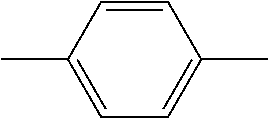H MeO H 129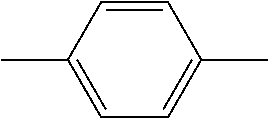Br MeO H 262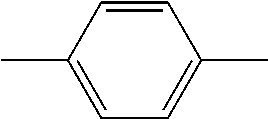MeO MeO H 343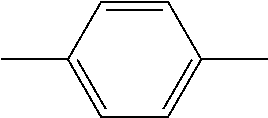H MeO MeO 130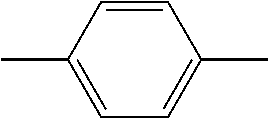H H H 131Br H H 357COOH MeO H LF3427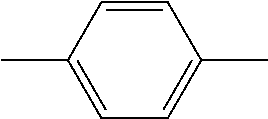forms together with R11 a phenyl ring MeO H 140H MeO H 143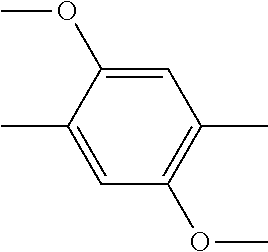H H H 173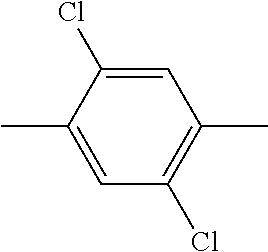H MeO H 174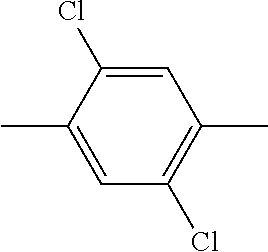H H H 194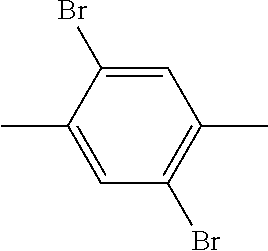H MeO H 335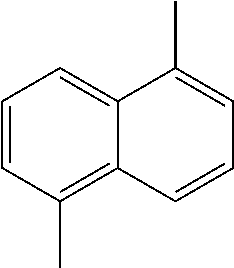H MeO H 156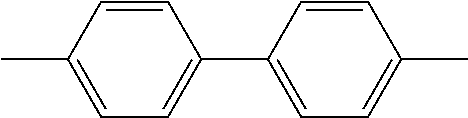H MeO H 157H H H 360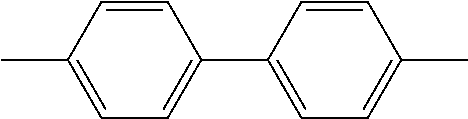COOH MeO H 183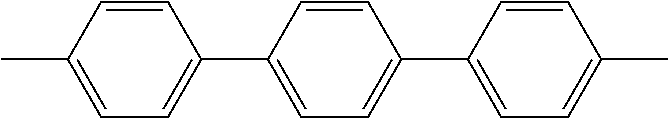H MeO H 184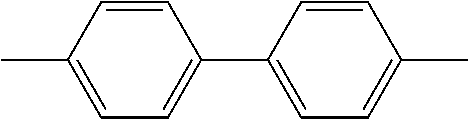H H H LF3375 —CH2—CH═CH—CH2— H MeO H LF 3376 —CH2—CH2—CH2— H MeO H 124 —CH2—CH2—CH2—CH2— H MeO H
• Best results have been obtained with the following bis-spiropyrans:
• Claim 4.
• Especially preferred is compound 127, which preparation is disclosed in Example 2.
• Further interesting compounds are:
• The indicator compounds of formula I or II are reversibly photochromic (Scheme 1).
• By virtue of its photochromic properties, the indicator compound can undergo photo-induced coloration by irradiation with photons of a specific energy range (conversion of the second isomeric form, thermodynamically more stable) into the first isomeric form (open form) the coloration being followed by a time- and temperature-dependent decoloration (conversion of the first isomeric form into the second isomeric form).
• The coloration of the indicator compound can take place at a defined time point, preferably, for example, immediately after printing onto a substrate, such as the packaging of a perishable material.
• In oligomeric spiropyrans there are at least two different metastable isomers. At least two distinct valence isomeric forms exist in each spiroaromatic unit of the oligomeric indicator. These isomeric forms are at least one colored open form, first isomeric form, and at least one colorless cyclic form (closed form or second isomeric form).
• Suitable active materials exhibit the following characteristics:
• (1) the system has at least one thermal process leading from one metastable state to one stable state, where the two states of the spiroaromatic compounds are characterized by a distinctly different color and/or any other measurable physical parameter such as luminescence, refraction index, conductivity and the like.
• (2) the stable state may be converted into the metastable state using one or any combination of stimuli, among others the following processes:
• a) photonic induction,
• b) thermal induction,
• c) pressure induction,
• d) electrical induction, or
• e) chemical induction; and
• (3) other than temperature and photoinduction (in the visible light range), the metastable state is substantially not affected by anyone or any combination of stimuli such as a) photo induction, b) piezo induction, c) electro induction, d) chemo induction.
• Photoinduction means that the initially colourless indicator is irradiated with light, preferably in the UV or near-UV range, as a result a reversible internal valence isomerisation from a colourless inactivated state to a coloured activated one is induced. A reverse discolouration process then proceeds at a rate that is time and temperature dependent.
• The metastable state may further be achieved by pressure induction. In this procedure, the matrix embedded with and/or atop the substance is passed between two bodies, such as metal rolls, which apply pressure onto the surface of the matrix thereby inducing the formation of the metastable state. By adjusting the time and pressure imparted by the bodies to the active material, it is possible to control the degree of conversion from a stable state to a metastable state in the TTI active matrix.
• The metastable state may be achieved by thermal induction. In this particular induction process, the matrix embedded with the substance to be induced is heated to temperatures normally below the melting point of said substance. The heat may be applied by any method known such as, but not limited to, a thermal transfer printing head. In one specific case, the heat is applied to the matrix while being passed through two heated metal rolls. In this case, the pressure applied to the surface is not capable itself of inducing the formation of the metastable state, but serves merely to ensure controlled thermal contact between the heaters and the sample. The metastable state is achieved as a result of the heat transfer from the heaters, i.e., the metal rolls, which are in contact with the matrix and the matrix itself.
• However, there may be instances where the use of any combination of pressure, light and thermal inductions may be desired or necessary. It is therefore, a further embodiment of the present invention, to achieve the metastable state of the substances to be used with the TTIs of the present invention, by a combination of stimuli.
• The active material of the present invention may be in the form of a crystal or a poly-crystalline powder, in which the forward and reverse reactions take place or alternatively may be in a form of any other condensed phase such as a glass, a polymer solution or attached to a polymer, or in the form of a liquid or a solution.
• In yet another aspect of the present invention, there is provided a method for the manufacture of a TTI comprising at least one of the spiroaromatic indicator compounds of the formula I or II in form of a pigment or a dye; said method comprising the steps of
• (a) introducing into a matrix or atop a matrix a dimeric or trimeric spiropyran indicator of the formula I or II as defined in claim 1 and
• (b) converting the spiropyran indicator from an original stable state into a metastable state by a process selected from photonic induction, thermal induction, pressure induction, electrical induction, or chemical induction,
• (c) optionally applying a protector film.
• (Claim 6)
• The converting step b may be effected immediately after step a) or later at any time.
• The original stable state and the metastable state is defined above (Scheme 1 above)
• The term “introducing into a matrix” means any form of admixing the TTI indicator into a matrix, for example, indicator-doping of the matrix, sol-gel embedment of the indicator in the matrix, embedment of the indicator as small crystallites, solid solution and the like.
• The matrix used in the present invention may be a polymer, an adhesive, all kinds of paper or cardboard, all kinds of printing media, metal, or any glass-like film.
• The matrix is also called substrate.
• Examples of printing media may be self-adhesive PP, cold lamination films, PVC films, PPpaper, glossy photo paper, vinyl sheets and the like; inkjet media.
• The matrix polymer is a high molecular weight organic material may be of natural or synthetic origin and generally has a molecular weight in the range of from 103 to 108 g/mol. It may be, for example, a natural resin or a drying oil, rubber or casein, or a modified natural material, such as chlorinated rubber, an oil-modified alkyd resin, viscose, a cellulose ether or ester, such as cellulose acetate, cellulose propionate, cellulose acetobutyrate or nitrocellulose, but especially a totally synthetic organic polymer (thermosetting plastics and thermoplastics), as are obtained by polymerisation, polycondensation or polyaddition, for example polyolefins, such as polyethylene, polypropylene or polyisobutylene, substituted polyolefins, such as polymerisation products of vinyl chloride, vinyl acetate, styrene, acrylonitrile, acrylic acid esters and/or methacrylic acid esters or butadiene, and copolymerisation products of the mentioned monomers, especially ABS or EVA. From the group of the polyaddition resins and polycondensation resins there may be mentioned the condensation products of formaldehyde with phenols, so-called phenoplasts, and the condensation products of formaldehyde with urea, thiourea and melamine, so-called aminoplasts, the polyesters used as surface-coating resins, either saturated, such as alkyd resins, or unsaturated, such as maleic resins, also linear polyesters and polyamides or silicones. The mentioned high molecular weight compounds may be present individually or in mixtures, in the form of plastic compositions or melts. They may also be present in the form of their monomers or in the polymerised state in dissolved form as film-forming agents or binders for surface-coatings or printing inks, such as boiled linseed oil, nitrocellulose, alkyd resins, melamine resins, urea-formaldehyde resins or acrylic resins.
• The term “introducing” means also printing. In this case, the TTI is transformed into a printable ink.
• The ink may directly be printed onto a matrix or directly onto the packaging material or label.
• Thus, the present invention further concerns a printing ink or printing ink concentrate, comprising at least one spiropyran indicator of the formula (I) or (II) as defined in claim 1; for manufacturing a time temperature indicator. (claim 9)
• Any of the printing methods known in the art can be used, e.g., ink jet printing, flexo printing, laser printing, thermo-transfer printing, pad printing, printing using cold lamination techniques, and the like.
• In another embodiment, the indicator compound is part of a thermal transfer (TTR) ink composition and is transferred to the printed surface by applying heat to the TTR layer. By means of a reference scale printed with the time-temperature integrator, absolute determination of quality grades is possible. The time-temperature integrator and the reference scale are advantageously arranged on a light-colored substrate in order to facilitate reading.
• It is possible to apply, preferably in black ink, further text (or information), such as an expiry date, product identification, weight, contents etc.
• The reference color may be changed as one means for changing the lifetime of the TTI.
• The time-temperature indicator may be covered with a protective film, designed to avoid photo recharging and/or photo bleaching.
• Either the TTI or the filter may be printed using cold lamination techniques or pad printing techniques.
• The protective film is, for example, a color filter, e.g. a yellow filter, which are permeable only to light having typical wavelengths that are longer than 430 nm.
• Suitable filters are disclosed in the International application EP2007/060987, filed Oct. 16, 2007. Disclosed therein is a composition comprising at least one ultraviolet light and/or visible light absorbing layer which is adhered to an underlying layer containing a photo-chromic colorant,
• which photo chromic colorant is activated by exposure to UV light to undergo a reversible color change, which color reversion occurs at a rate that is dependent on temperature,
wherein the light absorbing layer comprises a binder, from 1 to 60% by weight based on the total weight of the layer of an ultraviolet light absorber selected from the group consisting of hydroxyphenylbenzotriazole, benzophenone, benzoxazone, α-cyanoacrylate, oxanilide, tris-aryl-s-triazine, formamidine, cinnamate, malonate, benzilidene, salicylate and benzoate ultraviolet light absorbers.
• If desired an irreversible photo-sensitive indicator can be applied as tamper-proofing in the form of a covering over the time-temperature integrator. Suitable irreversible indicators include, for example, pyrrole derivatives, such as 2-phenyl-di(2-pyrrole)methane. Such a material turns irreversibly red when it is exposed to UV light.
• The invention further relates to a method of time temperature indication by converting the spiropyran indicator of the formula I or II as defined in claim 1 from an original stable state into a metastable state by a process selected from photonic induction, thermal induction, pressure induction, electrical induction, or chemical induction and detecting the time temperature dependent re-conversion from the metastable state to the original stable state. (claim 7)
• The time temperature detection may be achieved optically by detecting a change in an optical property (such as for example absorption, transmission, reflectivity) of the TTI device. For instance, a color change is determined either visually by comparing to a reference sample, or using a colorimeter or any colour reading or colour comparing technique. (claim 8)
• Preparation of Oligomeric Spirocompounds
• The photochromic spiropyran compounds of the present invention may be prepared according to synthetic routes known in the literature.
• The syntheses of bis-spirocompounds represented by formula I involve the process illustrated in Reactions A through E shown below and start from 2,3,3-trimethylindolenines which are commercially available (R5═H) or readily prepared by Fisher's reaction.
• Reaction A.
• Preparation of 5-substituted 2,3,3-trimethylindolenines of Formula III by Fisher's Reaction
• The reaction conditions are the standard ones described in the literature (Berman, E., Fox, R. E. and Thomson, F. D. Photochromic spiropyrans. I. The effect of substituents on the rate of ring closure. J. Am. Chem. Soc. 81, 1959, 5605-5608).
• Reaction B
• In Reaction B homobifunctional aromatic compounds may be prepared either by bromomethylation (Method I) or by radical bromination (Method II) of corresponding aromatic compounds.
• According to Method I an aromatic compound reacts with paraformaldehyde and hydrogen bromide in acetic acid in the presence of orthophosphoric acid under heating to give bifunctional compound represented by formula IY. The reaction conditions of the process are described in J. Am. Chem. Soc. 1992, 114: 6227-6238.
• Alternatively, compounds of formula IY may be prepared according to Method II using N-bromosuccinimide (NBS) in suitable non polar solvent, preferably benzene, chloroform, carbon tetrachloride, chlorobenzene, more preferably, benzene and chlorobenzene.
• Reaction C:
• In Reaction C substituted salicylaldehyde represented by formula Y (the substituents R1, R2, and R4 are the same as defined hereinabove) is dissolved in mixture of acetic acid and suitable organic solvent (such as dichloromethane, chloroform or the like) in ratio 1:1. The solution is treated with mixture of acetic and nitric acids under cooling with ice-water bath, to give after aqueous work up 5-nitrosubstituted salicylic aldehyde. Nitric acid concentration used in the process may be 100% or 70%, preferably 100%.
• Reaction D
• In reaction D indolenine of formula III reacts with bis-halomethyl compound represented by formula IY in an appropriate organic solvent (benzene, toluene, methylethylketone, acetonitrile, dioxane or a combination thereof) to give Fisher' base in the form of dihydrohalogenide. The reaction temperatures may be 80-120° C., preferably 85-90° C., reaction time may be about 10 h to about 3 days. The dihydrohalogenide of the Fisher' base YI is dissolved in dichloromethane and treated by aqueous solution of inorganic base (sodium hydroxide, sodium or potassium carbonate), to afford the free base YI, which is subjected to the next step without delay (because of the easiness of oxidation). Alternatively, the reaction may be carried out in the presence of organic (such as diisopropylethylamine, or other sterically hindered amines) or inorganic bases (such as potassium or sodium carbonates) to generate free base YI directly in the reaction mixture.
• Reaction E.
• In reaction E bis-spyropyran compounds may be formed from free Fisher's bases and the corresponding substituted salicylic aldehydes under reflux in suitable organic solvents (ethanol, acetonitrile, methylethylketone or dioxane)
• The preferred embodiments of the present invention are illustrated by the following examples, which are in no way intended to limit the scope of the present invention.
• EXAMPLES Example 1 Compound 156
• Step 1
• Reaction B:
• Biphenyl (15.4 g, 100 mmol) and paraformaldehyde (7.5 g, 250 mmol) were transferred into a 250 ml round bottom flask. HBr (33% in acetic acid, 100 ml, 579 mmol) and H3PO4 (20 ml) were added dropwise. The reaction mixture was stirred vigorously for 15 h at 80° C. under nitrogen. An additional aliquot of paraformaldehyde (2.5 g, 80 mmol) was added and the temperature raised to 120 C for 2 h. The reaction mixture was cooled to room temperature, the solids were filtered, washed with hexanes, recrystallized from benzene/hexane to afford 4,4′-Bis(bromomethyl)-1,1′biphenyl. Yield 5.4 g (15.9%)
• Step 2
• Step 2 involves the process described hereinabove as Reaction D:
• A solution of 4,4′-Bis(bromomethyl)-1,1′biphenyl (2.50 g, 7.4 mmol) and 2,3,3-trimethylindolenine (2.58 g, 16.1 mmol, 2.60 ml) in toluene (30 ml, AR) was stirred for 48 h at 80-85° C. An additional portion of the indolenine (1 g, 0.85 eq) was added and the reaction mixture was stirred for an additional 48 h. The reaction mixture was cooled to room temperature. A solid was filtered, washed with ether, THF, ether, affording 5.0 g of the crude 4,4′-bis((3,3-dimethyl-2-methyleneindolin-1-yl)methyl)-1,1′biphenyl as dihydrobromide.
• Step 3
• Step 3 involves the process described hereinabove as Reaction E.
• A solution of the 4,4′-bis((3,3-dimethyl-2-methyleneindolin-1-yl)methyl)-1,1′biphenyl dihydrobromide, (0.80 g, 1.6 mmol) in dichloromethane was treated with 5% NaOH under stirring for 0.5 h. The organic phase was separated, dried over Na2SO4, chromatographed on an alumina column in Hexane-CH2Cl2 (10-35%). Fractions containing free base were collected and the solvent was evaporated under reduced pressure (bath temp. 30° C., cooling under nitrogen). The self-crystallized free base was immediately suspended under heating in 50 ml ethanol containing a few drops of Et3N.
• 3-methoxy-5-nitrosalicylaldehyde (0.65 g, 3.3 mmol) was added to the free-base solution under heating and stirring. The reaction mixture was refluxed for 1 h, cooled to room temperature, and filtered through a glass filter. The solid product was washed with ethanol, triturated with Et3N (aq, 1%), washed with ethanol and hexane, and finally dried under vacuum to give bis-spiropyran 156. Yield 58%. The structure was confirmed by NMR and MS analysis.
• Example 2 Compound 127
• Step 1
• The process of Step 2 in example 1 was followed except that α,α′-dibromoxylene was used instead of 4,4′-bis(bromomethyl)-1,1′-biphenyl. The reaction mixture was stirred for 60 h. 1,4-bis((3,3-dimethyl-2-methyleneindolin-1-yl)methyl)-benzene as dihydrobromide was obtained with 69% yield.
• Step 2
• The process of Step 3 in example 1 was followed except that 1,4-bis((3,3-dimethyl-2-methyleneindolin-1-yl)methyl)benzene dihydrobromide was used instead of 4,4′-bis((3,3-dimethyl-2-methyleneindolin-1-yl)methyl)-1,1′-biphenyl dihydrobromide. Crude product was triturated with ethanol overnight, dried in vacuo to afford bis-spiropyran compound 127. Yield 67%.
• The structure was confirmed by NMR and MS analysis.
• Example 3 Compound 194
• Step 1
• Step 1 involves the process described hereinabove as Reaction B, Method II. 2,5-dibromo-p-xylene (10 g, 38 mmol) was dissolved in benzene (70 ml). Then NBS (14 g, 2.1 eq) and dibenzoyl peroxide (0.1 g, dried between two sheets of filter paper) were added and the mixture was refluxed under nitrogen. After 24 h, the succinimide was filtered off and the solvent was evaporated. The product was dissolved in chloroform, the solvent was partially evaporated and the crystals were formed under cooling. Crude product (6.7 g) was recrystallized from chloroform-hexane, giving rise to 5.0 g (31.2%) of pure 1,4-bis(dibromomethyl)-2,5-dibromobenzene. NMR spectrum conforms to the structure.
• Step 2
• The process of Step 2 in example 1 was followed with exception that 1,4-bis(dibromomethyl)-2,5-dibromobenzene was used instead of 4,4′-bis(bromomethyl)-1,1′biphenyl. The reaction mixture was filtered, washed with ether. Mother liquids and washings were joined, evaporated under reduced pressure, a residue was chromatographed on alumina (hexane-dichloromethane (0-30%) to give 1,4-bis((3,3-dimethyl-2-methyleneindolin-1-yl)methyl)-2,5-dibromo-benzene, which was subjected to the next step immediately.
• Step 3
• The process of Step 3 in example 1 was followed except that 1,4-bis((3,3-dimethyl-2-methyleneindolin-1-yl)methyl)-2,5-dibromo-benzene instead of 4,4′-bis((3,3-dimethyl-2-methyleneindolin-1-yl)methyl)-1,1′biphenyl. Yield 50%. NMR spectrum of the product conforms to the structure of bis-spirocompound FPSP194.
• Example 4 Compound 335
• Step 1
• The process of the Step 1 in example 3 was followed except that 1,5-dimethylnaphthalene (5.0 g, 32 mmol) was used instead of 2,5-dibromo-p-xylene. The reaction mixture was re-fluxed for 1 h (TLC monitoring: starting material disappeared after 0.5 h), cooled to room temperature; a precipitate was filtered, washed with benzene, suspended in 250 ml of water, washed with water for 45 min, filtered, dried giving rise to a crude product (˜10 g) which was crystallized from ethyl acetate to give 7.1 g (70.6%) of the pure bis-compound. NMR spectrum showed that the resulted product has the structure consistent with 1,5-dibromo-naphthalene.
• Step 2
• A mixture of 2,3,3-trimethylindolenine, 1,5-dibromomethylnaphthalene and potassium carbonate was heated at 90 C in 20 ml toluene for 48 h. Then the reaction mixture was filtered through alumina pad, alumina was washed with toluene. The joined filtrate and washings were evaporated under reduced pressure, a residue was chromatographed on alumina (Hexane-dichloromethane 0-10%). Fractions containing the product were collected and evaporated to give pure 1,5-bis((3,3-dimethyl-2-methyleneindolin-1-yl)methyl)-naphthalene which was subjected to the next step without delay.
• Step 3
• The process of Step 3 in example 1 was followed except that 1,5-bis((3,3-dimethyl-2-methyleneindolin-1-yl)methyl)-naphthalene was used instead of 4,4′-bis((3,3-dimethyl-2-methyleneindolin-1-yl)methyl)-1,1′biphenyl. The reaction mixture was refluxed overnight, cooled to room temperature, filtered, washed with ethanol, water, triturated with n-butanol, washed with ethanol, hexane, dried in vacuo, giving rise to light grey-greenish powder of FPSP335. Yield 81%. The NMR spectrum conforms to the structure.
• Example 5 Compound 183
• Step 1
• The process of Step 1 in Example 1 was followed except that p-terphenyl was used instead of 1,1′-biphenyl. Molar ratio—terphenyl:paraformaldehyde:HBr—1:6:8. The reaction mixture was heated at 80 C for 16 h under nitrogen. Then the temperature was raised to 120 C for 8 h, the reaction mixture was cooled to room temperature, solids were filtered, washed with acetone dried on a glass filter, to give crude 4,4″-bis-bromomethyl-[1,1′;4′,1″]terphenyl. The crude was repeatedly extracted with boiling toluene. The hot toluene solution was filtered and the product was crystallized under cooling to room temperature, filtered, dried in vacuum, giving rise to of 4,4″-bis-bromomethyl-[1,1′;4′,1″]terphenyl (23% yield (compound 181).
• Step 2
• A mixture of 2,3,3-tri-methyl-indolenine (4.29 g, 4.2 ml, 26.9 mmol), 4,4″-bis-bromomethyl-[1,1′;4′,1″]terphenyl (3.2 g, 7.69 mmol) and potassium carbonate (3.72 g. 26.9 mmol) in 50 ml of dioxane was heated at 90 C for 48 h, cooled to room temperature. The solvent was evaporated; a residue was partitioned between dichloromethane and 5% NaOH (aq), organic layer was separated, water layer was back extracted with dichloromethane, joined organic phases were dried over Na2SO4, concentrated under reduced pressure, chromatographed on alumina. Fractions containing bis-product (Rf=0.7, Slilca, dichloromethane-hexane—1:1) were collected, evaporated to dryness, to give crude free base 182 (yellow solid) which was subjected to the next step immediately.
• Step 3
• The process of Step 3 in example 1 was followed except that 4,4″-bis((3,3-dimethyl-2-methyleneindolin-1-yl)methyl)-[1,1,4′,1″]terphenyl was used instead of 4,4′-bis((3,3-dimethyl-2-methyleneindolin-1-yl)methyl)-1,1′biphenyl. The reaction mixture was cooled to room temperature, filtered through glass filter; solid product was washed with ethanol, water, triturated with ethanol under heating, dried in vacuo, to give bis spirocompound FPSP183. Yield 51.8%. The structure was confirmed by NMR and MS analysis.
• Example 6 Compound 357
• Step 1
• Step 1 involves the process described hereinabove as Reaction A.
• To a suspension of 4-hydrazinobenzoic acid (25 g, 164 mmol) in ethanol (500 ml) H2SO4 (8.8 ml, 16.12 g, 184 mmol) was added portionwise (under cooling with ice-water bath), then methyl isopropyl ketone (14.86 g, 18.46 ml, 173 mmol) was added and the reaction mixture was refluxed for 6 h, cooled to room temperature. After filtration, the solvent was evaporated, a residue was treated with 120 ml of sodium carbonate (sat), then pH was adjusted to 3-4 with acetic acid (glacial) and the mixture was extracted with dichloromethane 4×70 ml. Joined organic phases were dried over Na2SO4, passed through short silica column (elution dichloromethane-methanol-2-7%), fractions contained the product were collected, evaporated to dryness to afford solid reddish residue, which was re-crystallized from boiling toluene, washed with hexane, dried in vacuum, giving rise to 26.7 g (80% Yield) of 5-carboxy-2,3,3-trimethyl-indolenine. NMR spectrum conforms to the structure.
• Step 2
• A mixture of 5-carboxy-2,3,3-trimethyl-indolenine (4.0 g, 19.70 mmol) and α,α′-dibromoxylene (2.0 g, 7.58 mmol) in acetonitrile-toluene (60 ml, 1:2) was refluxed for 90 h. Then a brownish solid was filtered, washed with ether (2×20 ml), triturated with boiling toluene, following by hot filtration, washed with ether, to afford ˜5.8 g of crude material 356 as di-hydrobromide (pink powder). 2.4 g of the product 356 was dissolved in dichloromethane, treated with Na2CO3, and then pH of the water layer was adjusted to 3-4 by acetic acid. The organic phase was separated, water layer was extracted twice with dichloromethane, joined organic extracts were dried over Na2SO4, evaporated to dryness to afford 1,4-bis((5-carboxy-3,3-dimethyl-2-methyleneindolin-1-yl)methyl)-benzene (quantitative yield), which was subjected to the next step.
• Step 3
• The process of Step 3 in example 1 was followed except that 1,4-bis((5-carboxy-3,3-dimethyl-2-methyleneindolin-1-yl)methyl)benzene was used instead of 4,4′-bis((3,3-dimethyl-2-methyleneindolin-1-yl)methyl)-1,1′biphenyl. The reaction mixture was refluxed for 2 h in acetonitrile. Crude product was triturated with ethanol overnight, washed with ethanol, dried in vacuo, to afford 1.4 g (51.3%) SP357 as yellow green powder.
• Example 7 Compound 343
• Step 1
• Step 1 involves the process described hereinabove as Reaction C. 3,4-dimethoxy-salicylaldehyde (1.5 g, 8.23 mmol) was dissolved in the mixture of acetic acid (5 ml) and dichloromethane (5 ml). The solution was cooled to −10° C. (ice-water NaCl bath).
• A solution of fuming nitric acid (0.778 g, 0.512 ml, 1.5 eq) in 2 ml of acetic acid was added slowly by means of dropping funnel at such rate that the temperature was not exceed −5° C. After the reaction was completed (TLC monitoring), the mixture was poured into ice-water (100 ml) under vigorous stirring. The product precipitated was extracted with dichloromethane (3×20 ml), an organic phase was washed with 1M HCl (20 ml), dried over Na2SO4, passed through silica pad, evaporated to dryness giving rise to crude yellow product. The product was re-crystallized from ethanol, dried in vacuum. NMR spectrum conforms to the structure of 3,4-dimethoxy-5-nitro-salicylaldehyde.
• Step 2.
• To a suspension of 1,4-bis((3,3-dimethyl-2-methyleneindolin-1-yl)methyl)-benzene (0.31 g, 0.74 mmol) prepared as described in Example 2 (Step 2) in ethanol (45 ml) 3,4-dimethoxy-5-nitro-salicylaldehyde (0.336 g, 1.479 mmol) was added under stirring. The reaction mixture was refluxed for 2 h, cooled to room temperature, filtered, washed with ethanol, water, ethanol, giving rise to crude product FPSP343 (0.38 g, 51.4%), which was triturated with ethanol, dried in vacuo.
• The active crystalline materials of the TTIs were embedded in a suitable matrix including anti-foaming and anti-drying agents.
• The abovementioned materials displayed good resistance towards photobleaching. Results for five representative bis-spirocompounds (Scheme B) are presented in Table 1.
• The fading process of photoactivated compounds 1-5 was studied in a time frame of 150 hours. The measurements were performed at T=0° C. The fading process of TTIs, that were exposed to artificial light, followed a linear trend as a function of time (Table 1) with a moderate slope comparatively to the monomeric spiropyran included in the broad application. These results display a clear improvement both in terms of the quality and depth of the activated state's color and in the differentiation of the two coloured states, in comparison to prior art. Moreover, the life time of the activated state is increased and this feature is partially due to the enhanced photostability toward visible light of these compounds (see values for A colour intensity in Table 1).
•  TABLE 1 Fadinga Δ Colour intensityc Compound [Lab/h]b [Lab]b
 1 0.0904 10 2 0.0634 4 3α 0.0637 6 3β 0.0334 3 4 0.129 10 5 0.0352 (−2) aColour fading of the samples exposed to artificial light. bLab = (L2 + a2 + b2)0.5. cdecrease in colour intensity after exposure to artificial light for 150 hours.
• Stabilization Against Photobleaching
• Samples of the pigment were incorporated in identical water based ink, dispersed using a mill under the same conditions. The ink was printed on the same paper substance (LENETTA) and dried in an oven (30° C.) for 24 hrs. The samples were placed on 5 mm glass plates that served as a thermal reservoir and charged using the same light source (lamp 365 nm or LED 365-UV Light Emitting Diode (365 nm)). Two identical samples were prepared and charged from each ink. One system was placed in the dark at 0° C. while the other was exposed to filtered light (cutoff filter 455 nm) of a fluorescent lamp (“OSRAM” DULUX S G23, 900 lm, 11W/840), distance of 30 cm). The samples were measured using a colorimeter (Eye One GretagMacbeth). The CIE Lab values of the charged label that was kept in the dark were compared to the values of an identical label that was exposed to photobleaching light. As is evident from the following graphs, methoxy groups on the nitrophenyl group consistently reduce the photosensitivity of the colored species.
• Typically, the spiroaromatic compounds of the invention are incorporated into water based or solvent based ink (in some embodiments) prepared as follows.
• Preparation of the Ink Comprising Oligomeric Spiropyrans
• Water Based Ink Composition: 10% TTI
• Step 1. Polymer Matrix Preparation:
• 20 g of LS-16 (Ciba® GLASCOL® LS16—an aqueous microemulsion based on a carboxylated acrylic copolymer)
• 20 g of LS-20 (Ciba® GLASCOL® LS20—an aqueous microemulsion based on a carboxylated acrylic copolymer)
• 0.25 g of TEGO—TEGO® FOAMEX 845 defoamer emulsion of an organically modified polysiloxane, contains fumed silica)
• 0.1 g of triethanolamine (TEA)—stir for 1 min
• Step 2. Preparation of the Ink Sample
• 0.2 g of TTI
• 1.6 g of the Polymer matrix
• 0.4 g of water (HPLC grade)
• The mixture was dispersed on pulverisette (six cycles of 5 min at 600 rpm, twice: six cycles of 5 min at 800 rpm) to give the 10% TTI ink.
• Solvent Based Ink Composition: 10% TTI
• Step 1. Polyvinyl Butyrate (PVB) Varnish Preparation:
• 2 g PVB+8 g (10 ml) ethanol
• Stir for 2 h to afford a clear solution
• Step 2. Solvent Based Ink Concentrate Preparation
• 0.2 g of TTI
• 0.5 g of PVB varnish
• 0.2 g of ethanol
• 0.1 g of ethyl acetate
• Disperse on pulverisette (two cycles of 5 min at 600 rpm) to give an ink concentrate
• Step 3. Final Ink Preparation
• Add to the ink concentrate:
• 0.6 g of PVB varnish
• 0.4 g of ethanol
• 0.2 g of ethyl acetate
• Disperse on pulverisette (six cycles of 5 min at 600 rpm, then twice six cycles of 5 min at 800 rpm) to give the 10% TTI ink
•  Photobleaching Table at 0° C. The CIE Lab values of the charged label that was kept in the dark were compared to the values of an identical label that was exposed to photobleaching light.
 (L2 + a2 + b2)0.5 Charging Time, (L2 + a2 + b2)0.5
 Compound uncharged conditions hrs filter Dark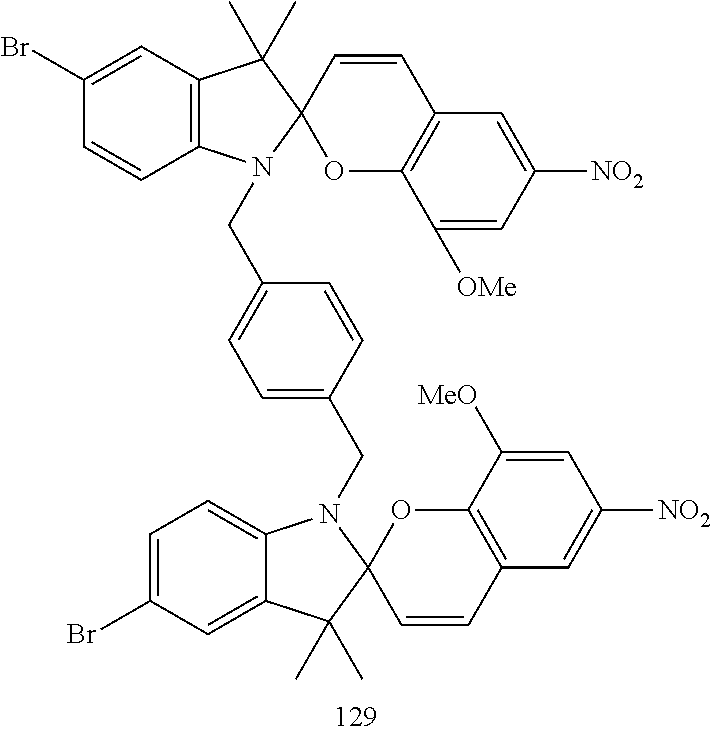89 3 min *Tube lamp 0  20  40  70 100 120 140 160 51 55 57 60 62 63 64 65 51 52 53 53 54 55 55 5595 3 min Tube lamp 0  25  50  75 100 125 150 57 60 62 63 65 70 72 57 60 60 61 61 62 63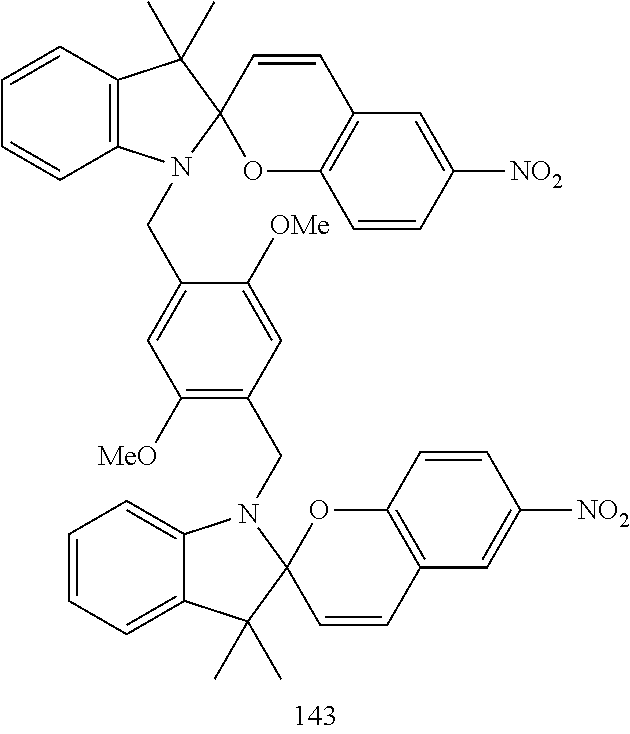87 5 min Tube lamp 0  25  50  75 100 57 59 61 63 68 57 55 55 56 56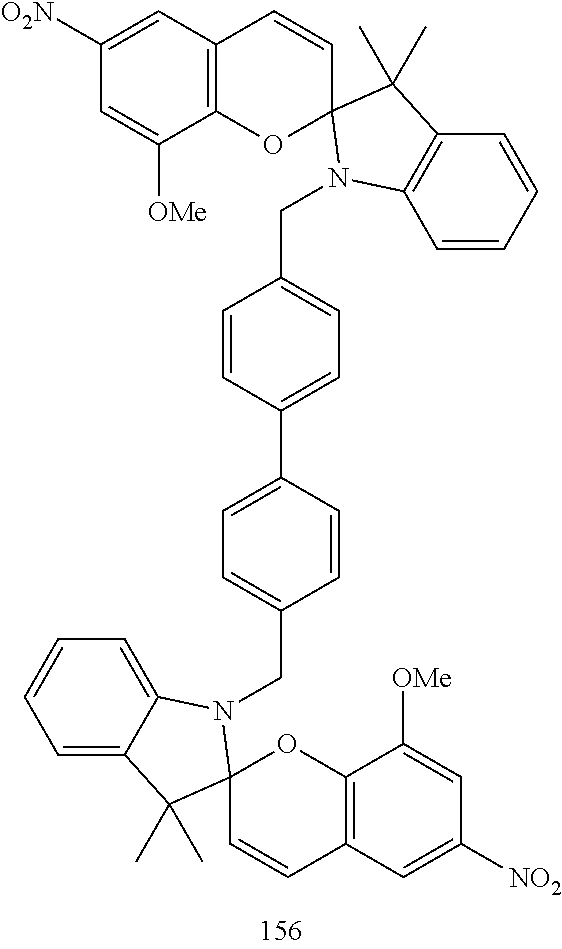84 3 min Tube lamp 0  25  50  75 100 125 150 175 200 225 250 275 48 49 49 49 49 50 50 51 52 52 52 53 48 49 49 49 49 49 49 49 50 50 50 5086 5 min Tube lamp 0  20  40  70 100 140 62 56 58 59 60 65 62 55 54 54 55 55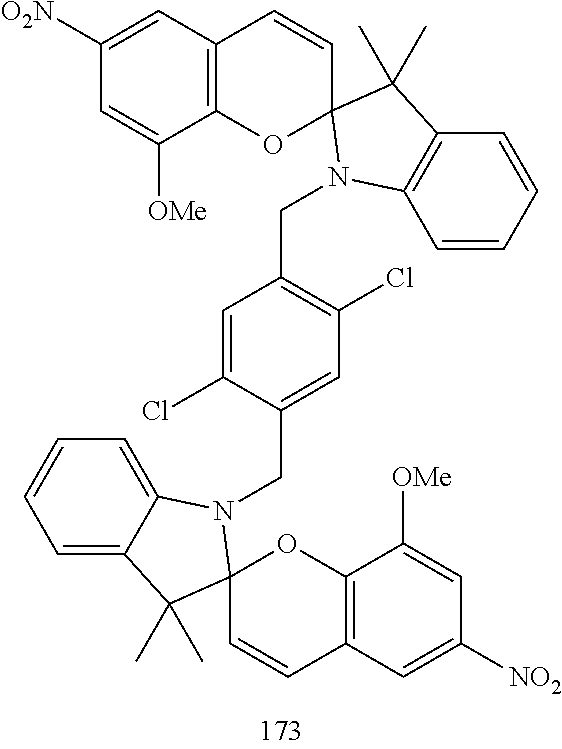93 2 min Tube lamp 0  10  20  50 57 62 64 72 57 62 63 67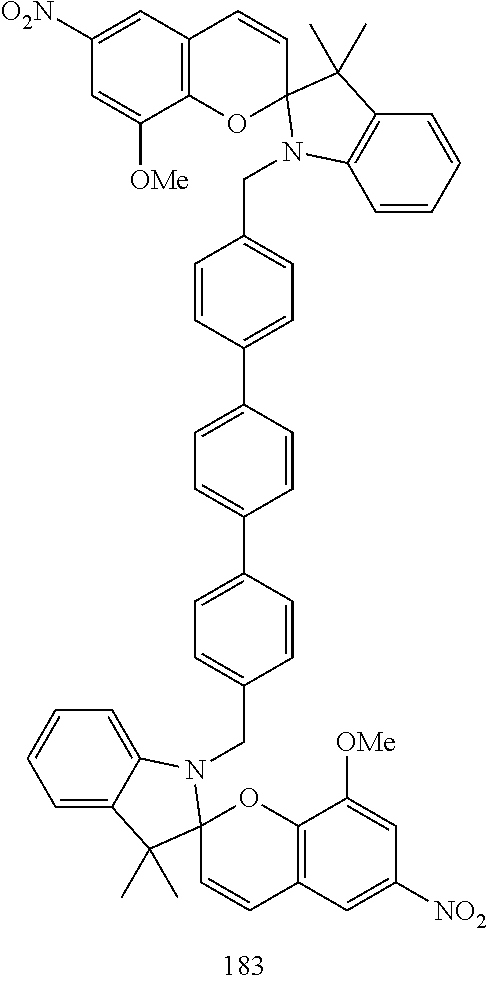87 2 min tube lamp 0  25  50  75 100 150 175 45 43 42 43 45 46 46 45 46 46 47 48 48 49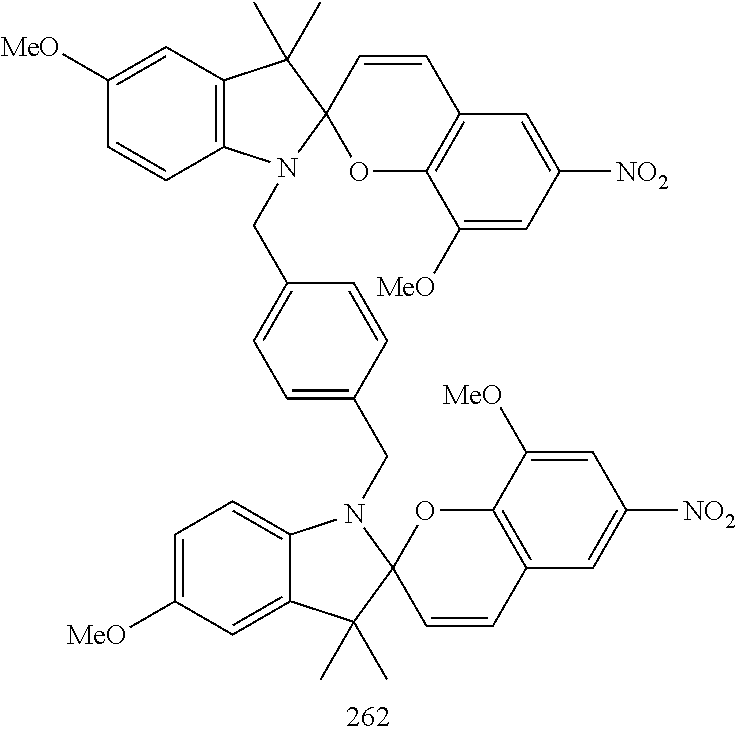65 15 sec **LED 365 0  20 120 53 51 53 53 51 52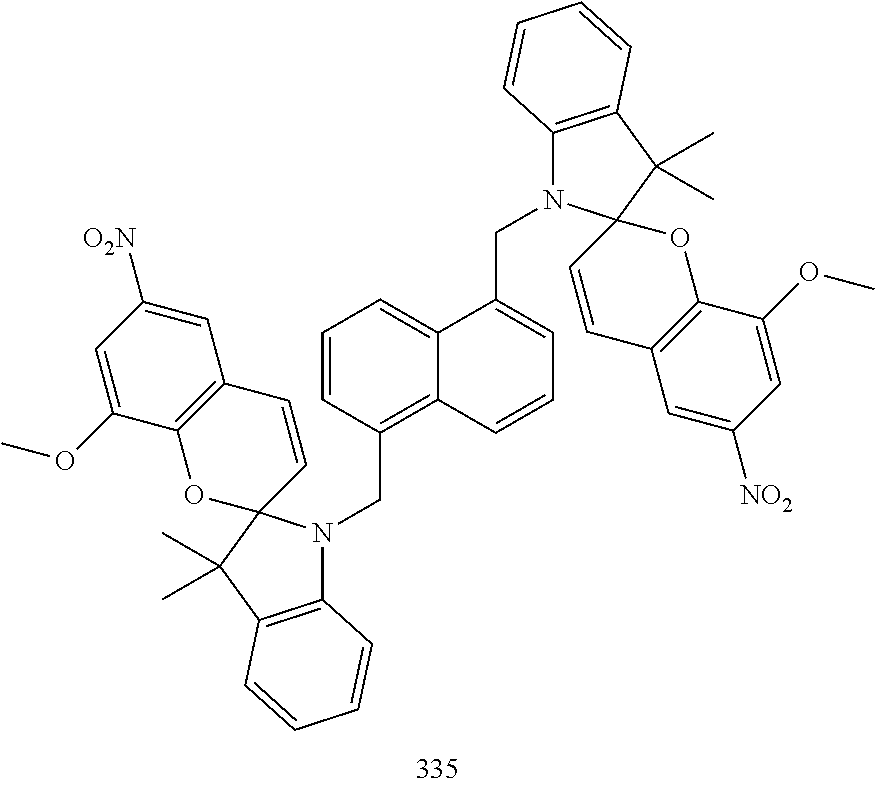65 15 sec LED 365 0  20  40  60  90 140 160 60 65 65 66 67 67 68 60 65 67 69 70 72 73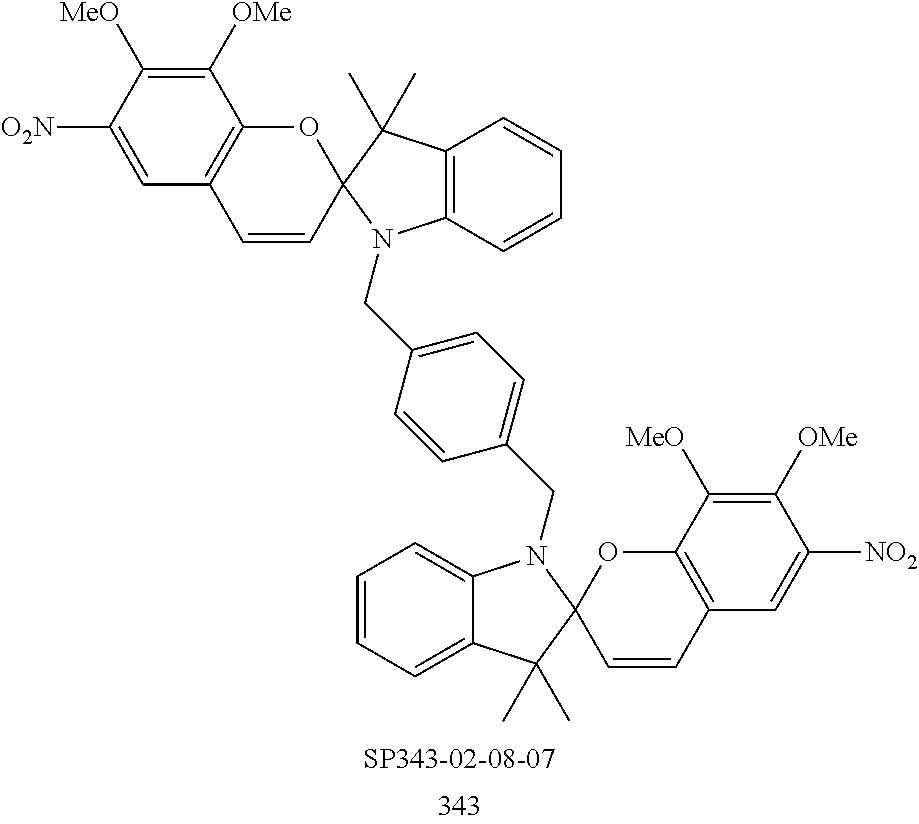88 15 sec LED 365 0  20  40  60  90 140 160 58 73 74 75 76 77 77 58 75 80 84 86 87 88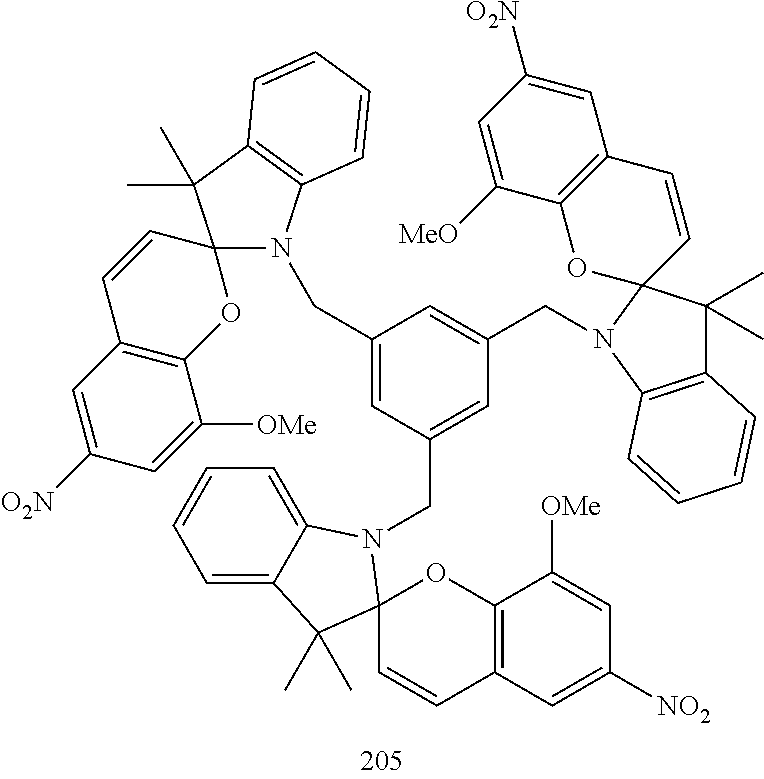76 15 sec LED 365 0  20  50  70  90 120 170 56 57 57 57 57 58 59 56 57 57 57 57 58 59 *Laboratory UV tube lamp VL—6.LC (6W-365 nm) **LED 365—UV Light Emitting Diode (365 nm)
• Kinetics of the fading processes are presented for two representatives of the oligomeric spiropyrans. The kinetic measurements were performed at various temperatures; the photo-activation of the oligomeric spiropyrans was carried out by irradiating the samples with either a 365 nm LED (about 300 mJ for compound 127) or a tube lamp (about 900 mJ for compound 140). The kinetic data shows that the fading process fits a bi-exponential time-temperature correlation.
• Kinetic measurements for the fading process of photo-activated compound 127
• 15 sec charging using LED 365 nm
•  (L2 + a2 + b2)0.5
 Time hrs 0° C. 5° C. 7° C. 10° C. 25° C.
 0 57 57 57 57 57 50 60 63 68 69 75 100 62 67 72 73 77 150 65 68 73 74 78 200 67 69 74 75 79 250 67 70 75 76 80 300 68 70 75 76 80 350 68 71 75 76 80 400 69 71 76 77 80
• Kinetic measurements for the fading process of photo-activated compound 140
• 15 sec charging using LED 365 nm
•  (L2 + a2 + b2)0.5
 Time hrs 0° C. 5° C. 10° C. 15° C. 25° C.
 0 57 57 57 57 57 50 60 61 62 85 91 100 60 63 64 91 91 150 61 67 68 91 91 200 61 67 68 92 250 61 68 69 93

## Claims (9)

1. A time temperature indicator comprising at least one dimeric or trimeric spiropyran indicator of the formula I or II
wherein
R1-R4 independently of one another is hydrogen, —C1-C6 alkoxy, halogen, CF3, —C1-C6 alkyl or —NO2;
R5 is hydrogen, halogen, —C1-C6 alkoxy, —COOH, —COO—C1-C6alkyl, —CF3 or phenyl;
R11 is hydrogen or R11 and R5 form together a phenyl ring;
Ra is —C1-C6 alkyl
Rb is —C1-C6 alkyl, or together with Ra form a 5-6 membered ring
2. The time-temperature indicator according to claim 1, comprising at least one trimeric spiropyran indicator of the formula II.
3. The time-temperature indicator according to claim 1, comprising at least one dimeric spiropyran indicator of the formula I.
4. The time-temperature indicator according to claim 3, wherein
R1 is hydrogen, —C1-C6 alkoxy, halogen, —C1-C6 alkyl or —NO2;
R2 is hydrogen or —C1-C6 alkoxy;
R3 is NO2 or halogen;
R4 is hydrogen, —C1-C6 alkoxy or halogen;
R5 is hydrogen, halogen, methoxy or —COOH
R11 is hydrogen,
Ra is methyl or ethyl.
Rb is methyl or ethyl.
5. The time-temperature indicator according to claim 1, wherein the at least one dimeric spiropyran indicator compound is selected from the group consisting of the following structural formulae
6. A method of manufacturing a time-temperature indicator according to claim 1 comprising at least one of the spiroaromatic indicator compounds of the formula I or II in form of a pigment or a dye; said method comprising the steps of
(a) introducing into a matrix or atop a matrix a dimeric or trimeric spiropyran indicator of the formula I or II and
(b) converting the spiropyran indicator from an original stable state into a metastable state by a process selected from photonic induction, thermal induction, pressure induction, electrical induction, or chemical induction,
(c) optionally applying a protector film.
7. A method of time temperature indication by converting the spiropyran indicator of the formula I or II according to claim 1 from an original stable state into a metastable state by a process selected from photonic induction, thermal induction, pressure induction, electrical induction, or chemical induction and detecting the time temperature dependent re-conversion from the metastable state to the original stable state.
8. The method of claim 7, wherein a color change is detected based on the color difference between said metastable and original state.
9. A printing ink or printing ink concentrate for manufacturing a time temperature indicator comprising at least one spiropyran indicator of the formula (I) or (II)
wherein
R1-R4 independently of one another is hydrogen, —C1-C6 alkoxy, halogen, CF3, —C1-C6 alkyl or —NO2;
R5 is hydrogen, halogen, —C1-C6 alkoxy, —COOH, —COO—C1-C6alkyl, —CF3 or phenyl;
R11 is hydrogen or R11 and R5 form together a phenyl ring;
Ra is —C1-C6 alkyl
Rb is —C1-C6 alkyl, or together with Ra form a 5-6 membered ring
US12/523,110 2007-01-22 2008-01-14 Time-temperature indicator based on oligomeric spiroaromatics Abandoned US20110059545A1 (en)

## Applications Claiming Priority (3)

Application Number Priority Date Filing Date Title
EP07100873 2007-01-22
EP07100873.4 2007-01-22
PCT/EP2008/050323 WO2008090045A1 (en) 2007-01-22 2008-01-14 Time-temperature indicator based on oligomeric spiroaromatics

## Publications (1)

Publication Number Publication Date
US20110059545A1 true US20110059545A1 (en) 2011-03-10

# Family

## Family Applications (1)

Application Number Title Priority Date Filing Date
US12/523,110 Abandoned US20110059545A1 (en) 2007-01-22 2008-01-14 Time-temperature indicator based on oligomeric spiroaromatics

## Country Status (6)

US (1) US20110059545A1 (en)
EP (1) EP2121870A1 (en)
JP (1) JP5010688B2 (en)
KR (1) KR20100014829A (en)
CN (1) CN101646744A (en)
WO (1) WO2008090045A1 (en)

## Cited By (4)

* Cited by examiner, † Cited by third party
Publication number Priority date Publication date Assignee Title
US20110092364A1 (en) * 2009-10-21 2011-04-21 Xerox Corporation Oligomeric spiropyrans for erasable medium applications
US8287776B2 (en) 2009-01-08 2012-10-16 Basf Se Preparation of a photochromic ink
CN114401972A (en) * 2019-10-17 2022-04-26 株式会社德山 Photochromic compound and curable composition containing same
WO2022161960A1 (en) 2021-01-29 2022-08-04 Basf Se A method of marking fuels

## Families Citing this family (8)

* Cited by examiner, † Cited by third party
Publication number Priority date Publication date Assignee Title
EP2385947A1 (en) 2009-01-08 2011-11-16 Basf Se Time temperature indicator comprising indolenin based spiropyrans
US20110287549A1 (en) 2009-02-12 2011-11-24 Freshpoint Holdings Sa Time temperature indicator based on thioalkyl and thioaryl substituted spiroaromatics
ES2376559B1 (en) 2011-04-11 2013-01-24 Universidad Pública de Navarra DEVICE FOR MONITORING TIME AND TEMPERATURE CONDITIONS.
CN103175625B (en) * 2013-02-25 2015-07-29 上海大学 Based on the optic temperature sensor of temperature sensitive cyclodextrin, its preparation method and application
CN103725103A (en) * 2013-06-19 2014-04-16 王斌 Time-temperature indicator oil
CN105793682B (en) * 2013-10-10 2018-12-28 麦克马斯特大学 Temperature changes indicator and its manufacturing method
GB2534113B (en) * 2014-09-12 2020-11-18 Domino Uk Ltd Ink composition
CN106671639A (en) * 2017-01-25 2017-05-17 上海先幻新材料科技有限公司 Secure file containing friction-modulated luminescent compound

## Citations (1)

* Cited by examiner, † Cited by third party
Publication number Priority date Publication date Assignee Title
US20070172951A1 (en) * 2004-02-02 2007-07-26 Yoav Levy Time-temperature indicator based on valence isomerizations

## Patent Citations (1)

* Cited by examiner, † Cited by third party
Publication number Priority date Publication date Assignee Title
US20070172951A1 (en) * 2004-02-02 2007-07-26 Yoav Levy Time-temperature indicator based on valence isomerizations

## Cited By (5)

* Cited by examiner, † Cited by third party
Publication number Priority date Publication date Assignee Title
US8287776B2 (en) 2009-01-08 2012-10-16 Basf Se Preparation of a photochromic ink
US20110092364A1 (en) * 2009-10-21 2011-04-21 Xerox Corporation Oligomeric spiropyrans for erasable medium applications
US8603944B2 (en) * 2009-10-21 2013-12-10 Xerox Corporation Oligomeric spiropyrans for erasable medium applications
CN114401972A (en) * 2019-10-17 2022-04-26 株式会社德山 Photochromic compound and curable composition containing same
WO2022161960A1 (en) 2021-01-29 2022-08-04 Basf Se A method of marking fuels

## Also Published As

Publication number Publication date
JP2010518360A (en) 2010-05-27
WO2008090045A1 (en) 2008-07-31
JP5010688B2 (en) 2012-08-29
KR20100014829A (en) 2010-02-11
CN101646744A (en) 2010-02-10
EP2121870A1 (en) 2009-11-25

## Similar Documents

Publication Publication Date Title
US20110059545A1 (en) Time-temperature indicator based on oligomeric spiroaromatics
EP2102307B1 (en) Photostabilized time temperature indicator
US8277749B2 (en) Time-temperature indicator based on valence isomerizations
TWI249561B (en) Stabilized adhesive compositions containing highly soluble, red-shifted, photostable benzotriazole UV absorbers and laminated articles derived therefrom
US20100043695A1 (en) Color changing indicator
CN101652448B (en) TTI indicator with balanced photochemical processes
JP2008213487A (en) Imaging system
US5097034A (en) Triarylmethane color formers
EP2291382B1 (en) Time temperature indicator comprising indolenin based spiropyrans containing a n-acetylamido or n- acetylester side chain
US20110269242A1 (en) Time temperature indicator comprising indolenin based spiropyrans
CA2051320A1 (en) Pressure-sensitive or heat-sensitive recording material
US20110287549A1 (en) Time temperature indicator based on thioalkyl and thioaryl substituted spiroaromatics
US3967835A (en) Pressure-sensitive copying material
US4897223A (en) Tri-phenyl methyl color-forming agents
US4073614A (en) Mixture of benzoxazines and benzodioxanes which may be used as color formers
EP0145225B1 (en) Record material
US4074050A (en) Diphenyl-1,3-benzoxazine-2-ones
US3896126A (en) 2-oxo-3 spiropyran quinoxalines
JP7338901B2 (en) DIARYLETHENE COMPOUND, TEMPERATURE INDICATOR, TEMPERATURE INDICATION DEVICE AND PACKAGE

## Legal Events

Date Code Title Description
AS Assignment

Owner name: FRESHPOINT HOLDINGS S.A., SWITZERLAND

Free format text: ASSIGNMENT OF ASSIGNORS INTEREST;ASSIGNORS:SALMAN, HUSEIN;TENETOV, ELENA;FEILER, LEONHARD;AND OTHERS;SIGNING DATES FROM 20090709 TO 20090720;REEL/FRAME:025430/0082

STCB Information on status: application discontinuation

Free format text: ABANDONED -- FAILURE TO RESPOND TO AN OFFICE ACTION